# Solved Problems: Mechanics Of Fluid (Fluid Kinematics And Dynamics)

Solved Problems: Mechanics Of Fluid (Fluid Kinematics And Dynamics)

Problem 1

A U-Tube manometer is used to measure the pressure of water in a pipe line, which is in excess of atmospheric pressure. The right limb of the manometer contains water and mercury is in the left limb. Determine the pressure of water in the main line, if the difference in level of mercury in the limbs U. U tube is 10 cm and the free surface of mercury is in level with over the centre of the pipe. If the pressure of water in pipe line is reduced to 9810 N/m2, Calculate the new difference in the level of mercury. Sketch the arrangement in both cases.

Given,

Difference of mercury  = 10 cm = 0.1 m.

Let PA = pr of water in pipe line (ie, at point A)

The point B and C lie on the same horizontal line. Hence pressure at B should be equal to pressure at C.

But pressure at B = Pressure at A and Pressure due to 10 cm (or) 0.1m of water.

=PA +p ×g ×h

where , P = 1000kg/m3 and h = 0.1 m

=PA  +1000 ×9.81×0.1

=PA  +981N / m 2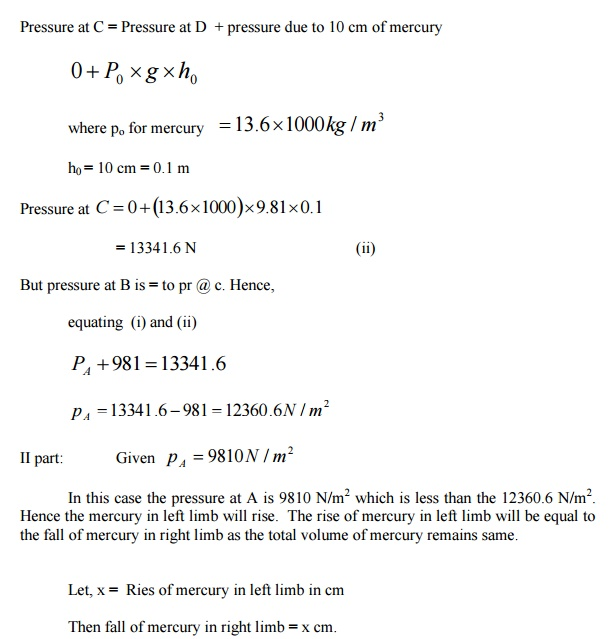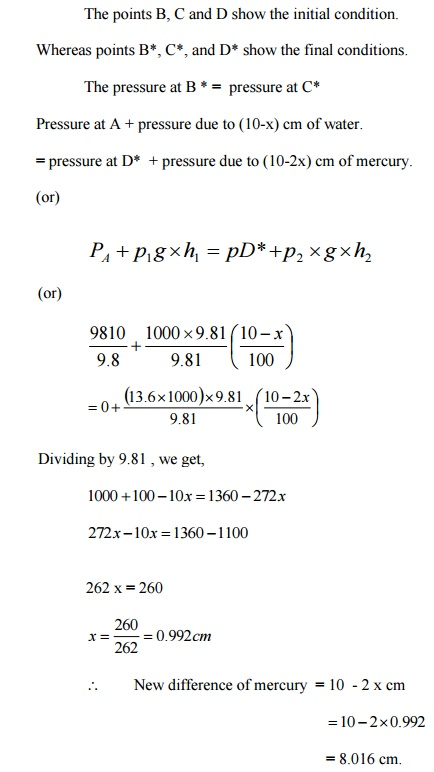Problem 2:

A differential manometer is connected at the two points A and B of two pipes a shown in figure. The pipe A contains a liquid of sp. Gr = 1.5 while pipe b contains a liquid of sp . gr = 0.9 .The pressures at A and B are 1kgf/cmrespectively. Find  the  difference  in mercury level  in  the  differential manometer.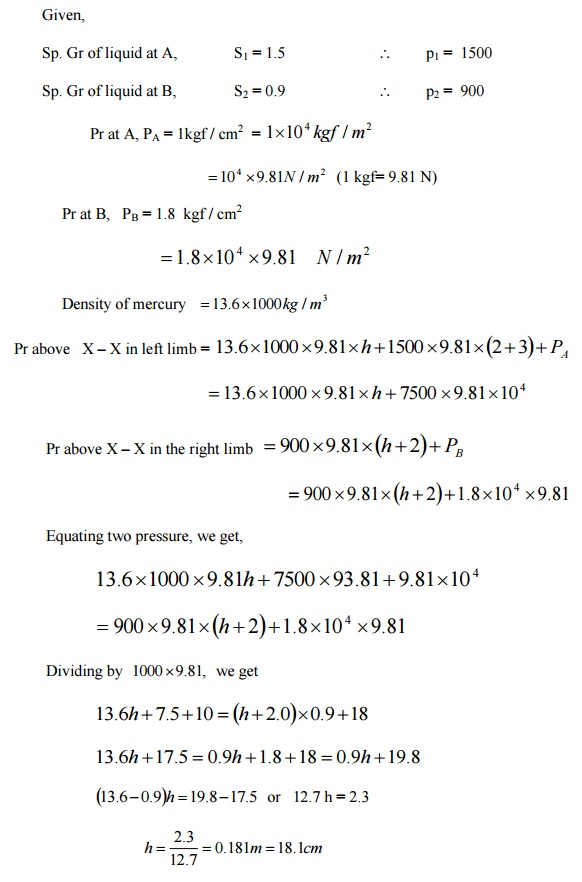Problem 3

A vertical sluice gate is used to cover an opening in a dam. The opening is 2m wide and 1.2m high. On the upstream of the gate, the liquid of sp. Gr

1.45, lies upto a height of 1.5m above the top of the gate, whereas on the downstream side the water is available upto a height touching the top of the gate. Find the resultant force acting on the gate and position of centre of pressure. Find also the force acting horizontally at the top of the gate and posiotn of centre of pressure. Find also the force acting horizontally at the top of the gate which is capable of opening it. Assume the gate is hinged at the bottom.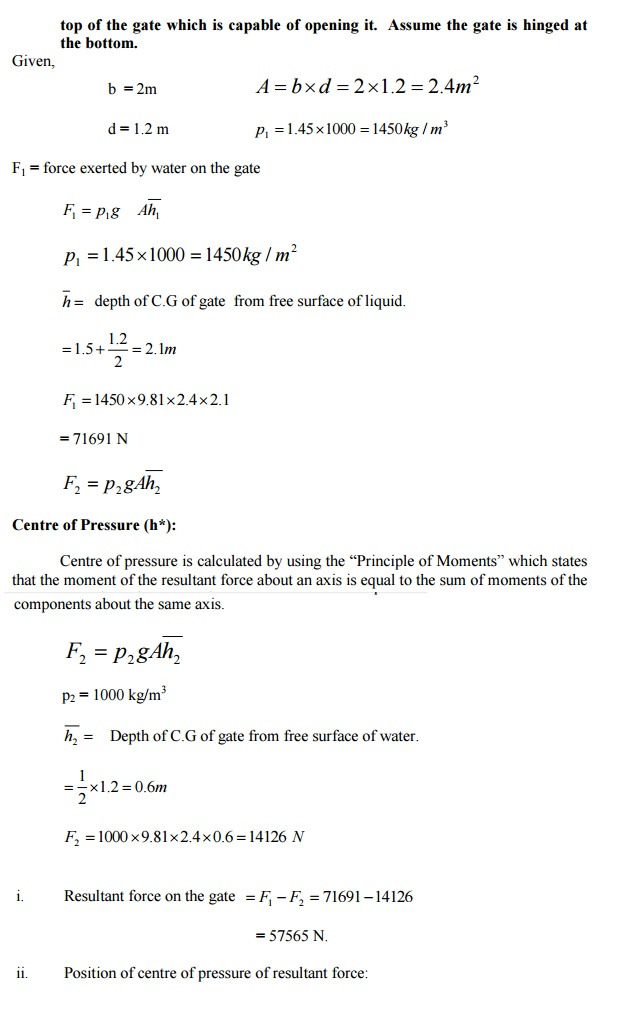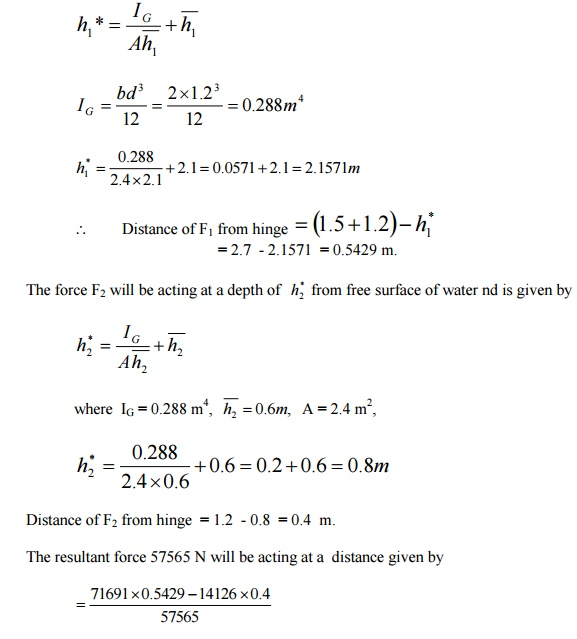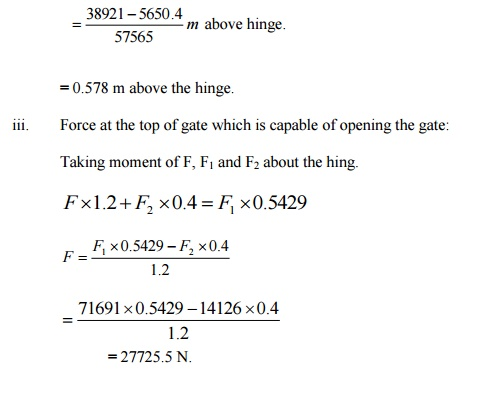Problem 4

Find the density of a metallic body which floats at the interface of mercury of sp. Gr 13.6 and water such that 40% of its volume is sub-merged in

mercury and 60% in water.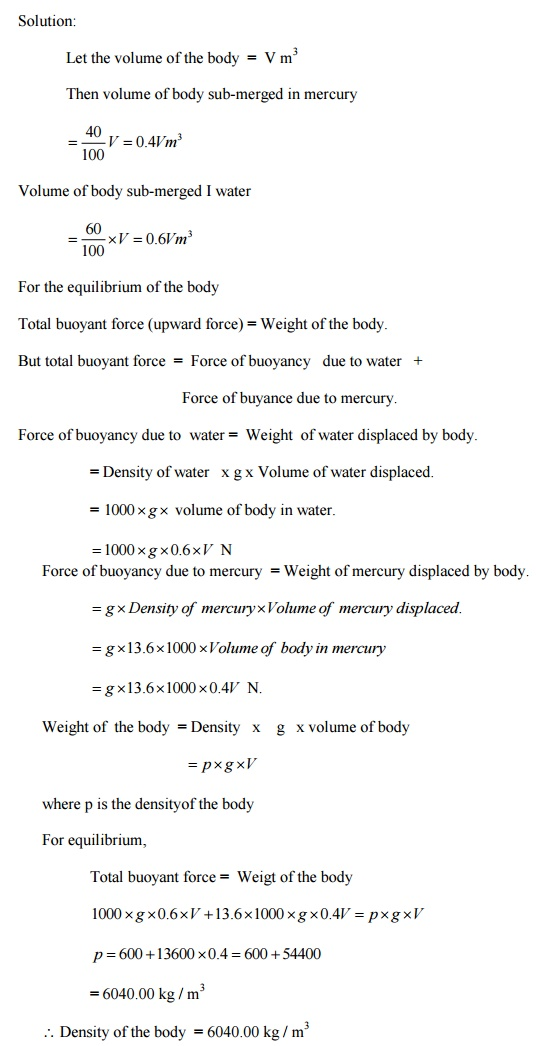5.     A solid cylinder of diameter 4.0 m has a height of 3m. Find the meta – centric height of the cylinder when it is floating in water with its axis vertical. The sp gr of the cylinder -0.6 .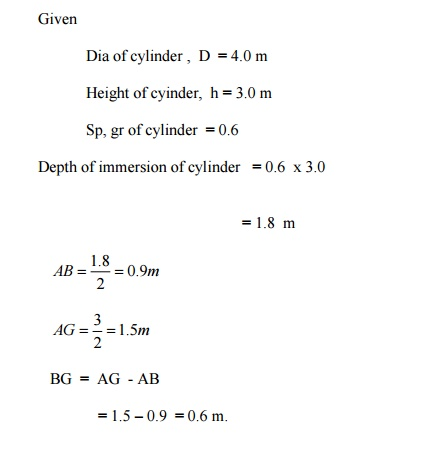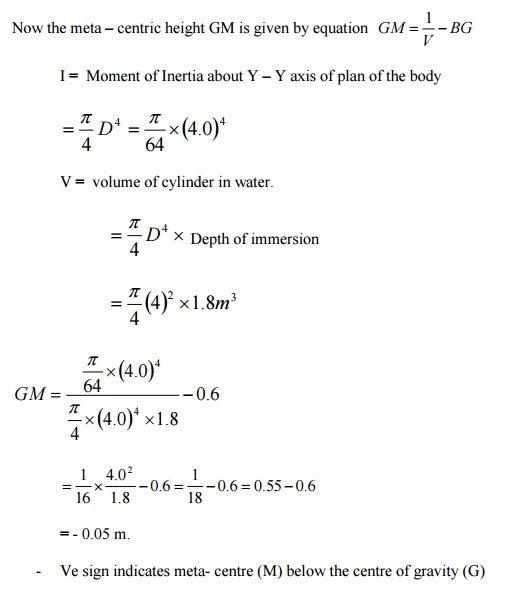Problem 6

A wooden cylinder of sp. Gr = 0.6 and circular in cross -section is required to float in oil (sp.gr = 0.90) . Find the L/D ratio for the cylinder to float with its longitudinal axis vertical in oil, where L is the height of cylinder and D is

its diameters.

Solution:

Dia of cylinder  =  D

Height of cylinder  = L

Sp gr of cylinder, S1 = 0.6

Sp gr of oil, S2 = 0.9

Let, depth of cylinder immersed in oil = h

Buoyancy principle

Weight of cylinder = Weight of oil dispersed.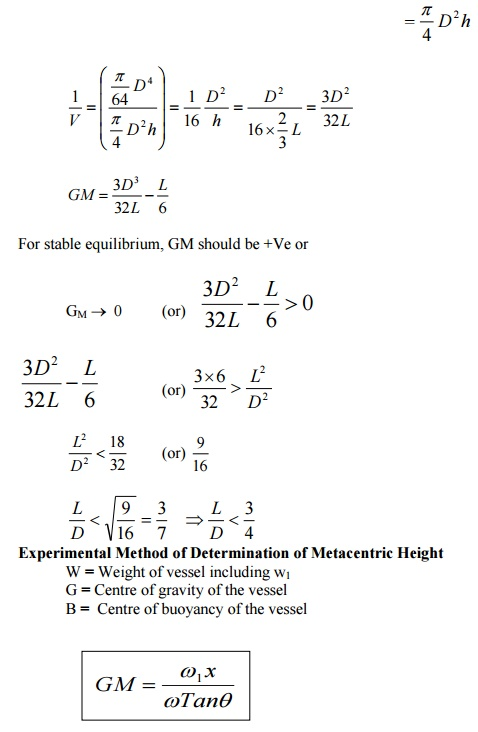Problem 7

In  a  two  -two  dimensional  incompressible  flow, the fluid velocity

components are given by u = x -4y and v= – y -4x.

show that velocity potential exists and determine its form. Find also the stream function.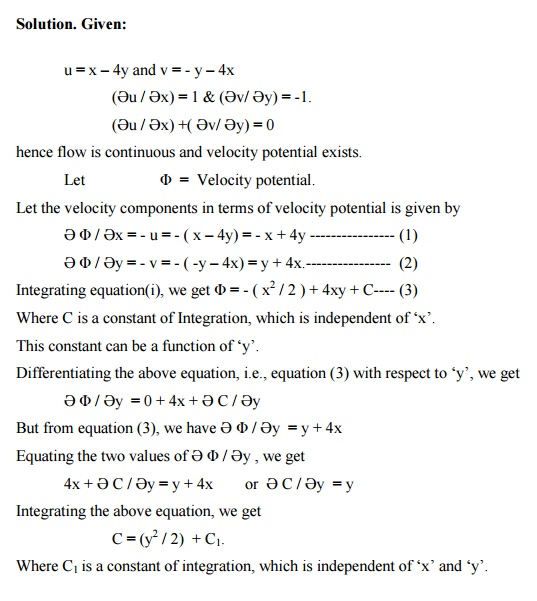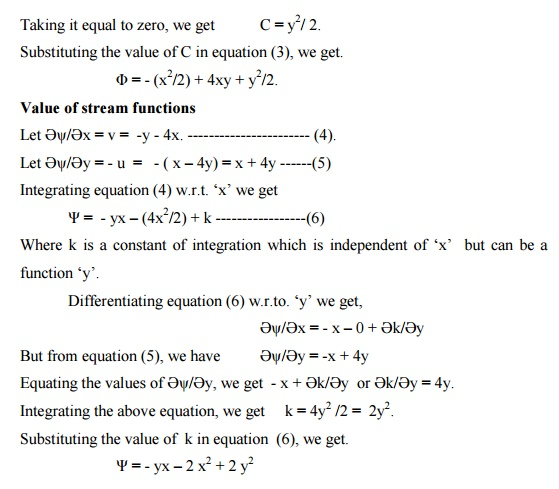SECTION 21.A pipe through which water is flowing is having diameters 20 cm and 10 cm at the cross-sections 1 and 2 respectively. The velocity of water at section 1 is given 4 m/s. Find the velocity head at sections 1 and 2 and also rate of discharge.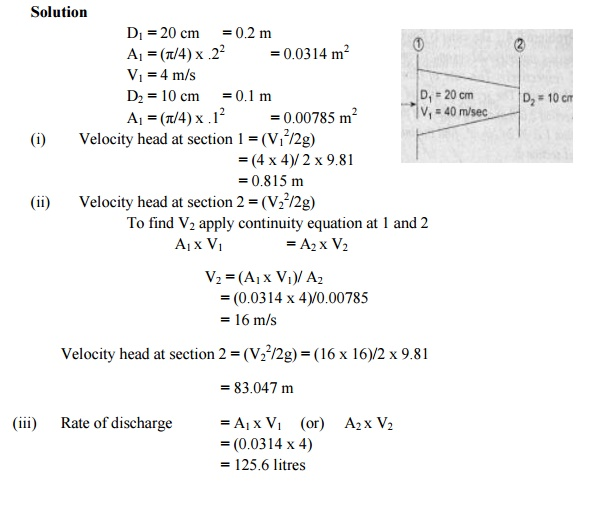2.The water is flowing through a pipe having diameter 20 cm and 10 cm at sections 1 and 2 respectively. The rate of flow through pipe is 35 litres/s. The section 1 is 6 m above datum and section 2 is 4 m above datum. If the pressure at section 1 is 39.24 N/cm2, find the intensity of pressure at section 2.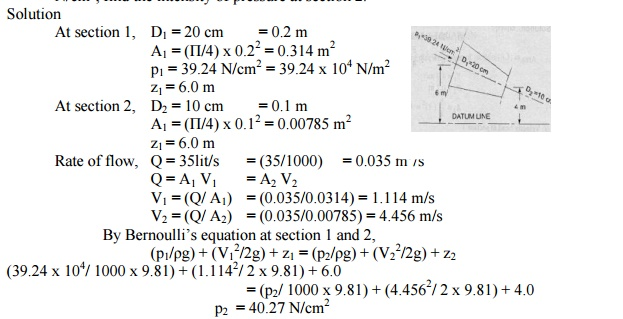3.Water is flowing through a pipe having diameter 300 mm and 200 mm at the bottom and upper end respectively. The intensity of pressure at the bottom end is 24.525 N/cm2 and the pressure at the upper end is 9.81 N/cm2. Determine the difference in datum head if the rate of flow through pipe is 40 lit/s.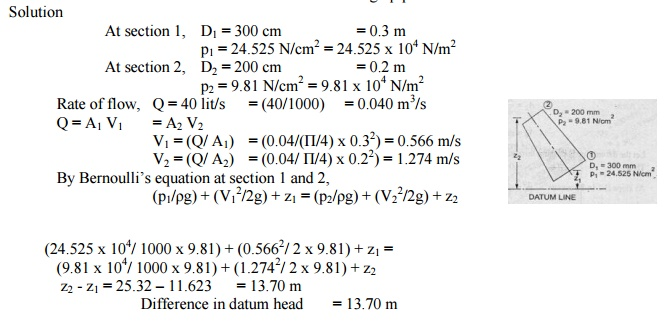4.In a two dimensional incompressible flow the fluid velocity components are given by u = x  4y and v = -y  4x, Where u and v are x and y components of flow velocity. Show that the flow satisfies the continuity equation and obtain the expression for stream function. If the flow is potential, obtain also the expression for the velocity potential.5.The stream function and velocity potential for a flow are given by ? = 2xy and ? = x2  y2. Show that the conditions for continuity and irrotational flow are satisfied. Solution:From the properties of Stream function, the existence  of stream function shows the possible  case   of  flow       and  if  it  satisfies  Laplace    equation,  then the  flow  is irrotational.

5. A 250 mm diameter pipe carries oil of specific gravity 0.9 at a velocity of 3 m/s. At another section the diameter is 200 mm. Find the velocity at this section and the mass rate of flow of oil.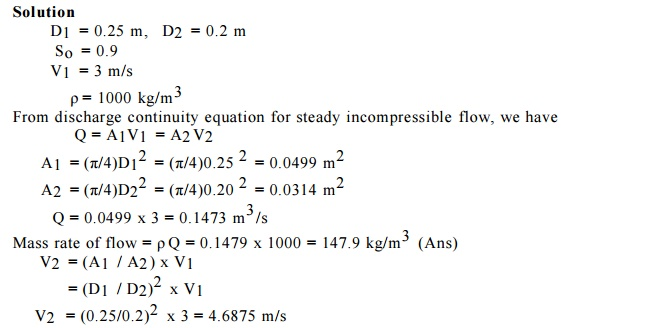9.A 30 cm x 15 cm venturimeter is inserted in a vertical pipe carring water, flowing in a upward direction. A differential mercury manometer connected to the inlet and throat gives a reading of 20 cm. Find the discharge. Take Cd = 0.98.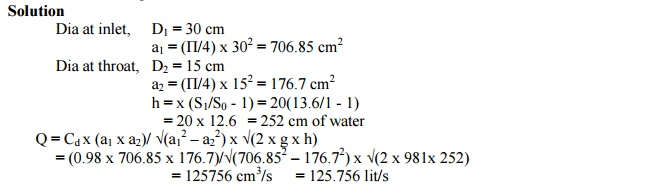It states that in a steady, ideal flow of an incompressible fluid, the total energy at any point of the fluid is constant. The total energy consists of pressure energy, kinetic energy and potential energy or datum energy.

Assumptions made in the derivation   of   Bernoulli’s   equation

(i). The fluid is ideal, i.e., Viscosity is zero.  (ii). The flow is steady

(iii). Te flow is incompressible.                (iv). The flow is irrotational.

Bernoulli’s  theorem for steady flow of an incompressible fluid.

It states that in a steady, ideal flow of an incompressible fluid, the total energy at any point of the fluid is constant. The total energy consists of pressure energy, kinetic

energy and potential energy or datum energy. These energies per unit weight of the

fluid are:

Pressure Energy                    =   p   /   ?g

Kinetic energy                       = v2 / 2g

Datum Energy                      = z

The                                             mathematically,   Bernoulli’s   theo

(p/w) + (v2 / 2g) + z = Constant.

Problem 1

Water is flowing through a pipe of 5 cm diameter under a pressure of 29.43 N/cm2

(gauge) and with mean velocity of 2.0 m/s. find the total head or total energy per unit weight of the water at cross -section, which is 5 cm above the datum line.

Diameter of the pipe                5 cm = 0.5 m.

Pressure      ? = 29.43 N/cm2 = 29.23 N/m2

`        velocity,      v        = 2.0 m/s.

datum head z        = 5 m

pressure head                 =   (p/   ?g) 4 / (2X9=(.81)) 29=30.m43X10

kinetic head          = (v2/ 2g) = ( 2X2/(2X9.81)) = 0.204 m

=   (p/+ (v(?g))/2g)+z

= 30 + 0.204 + 5 = 35.204m

Problem 2

Water is flowing through two different pipes, to which an inverted differential manometer having an oil of sp. Gr 0.8 is connected the pressure head in the pipe A is 2 m of water, find the pressure in the pipe B for the manometer readings.Problem 3

The diameters of a pipe at the sections 1 and 2 are 10 cm and 15 cm respectively. Find the discharge through the pipe if the velocity of water flowing through the pipe section 1 is 5 m/s. determine also the velocity at section 2.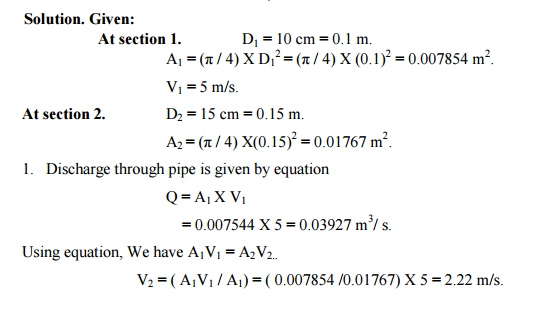Problem 4

A pitot -static tube is used to measure the velocity of water in a pipe. The stagnation pressure head is 6mm and static pressure head is 5m. Calculate the velocity of flow assuming the co-efficient of tube equal to 0.98.Problem 5

A sub-marine moves horizontally in a sea and has its axis 15 m below the surface of water. A pitot tube properly placed just in front of the sub-marine and along its axis connected to the two limbs of a U -tube containing mercury. The difference of mercury level is found to be 170 mm. find the speed of the sub-marine knowing that the sp.gr. of mercury is 13.6 and that of sea-water is 1.026 with respect fresh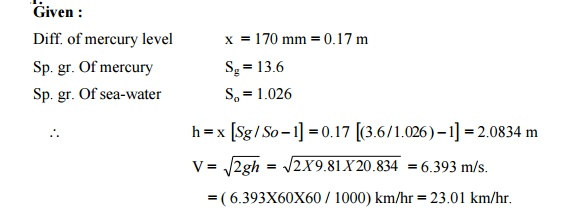Problem 6

The water is flowing through a pipe having diameters 20 cm and 10 cm at sections 1 and 2 respectively. The rate of flow through pipe is 35 lit/sec. the section 1 is 6m above datum. If the pressure at section 2 is 4m above the datum. If the pressure at section 1 is 39.24 N/cm2, find the intensity of pressure at section 2.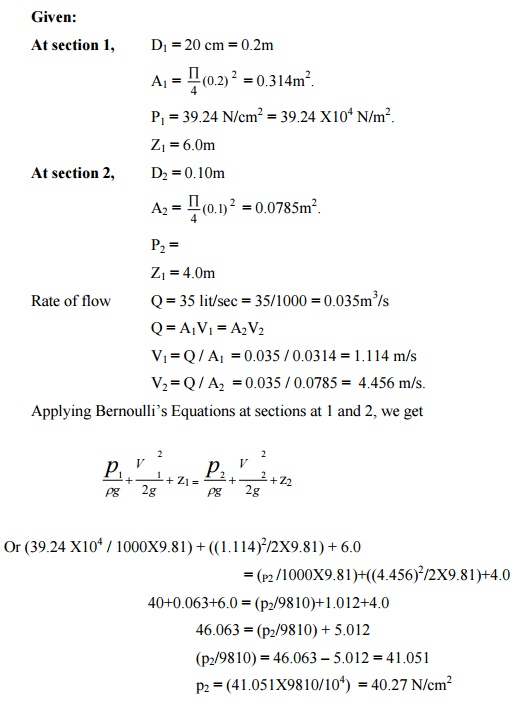Problem 7

In a vertical pipe conveying oil of specific gravity 0.8, two pressure gauges have been installed at A and B where the diameters are 16 cm and 8 cm respectively. A is 2 m above B. the pressure gauge readings have shown that the pressure at B is greater than at A by 0.981 N/cm2. Neglecting all losses, calculate the flow rate. If the gauges at A and B are replaced by tubes filled with the same liquid and connected to a U – tube containing mercury, calculate the difference of level of mercury in the two limbs of the U-tube.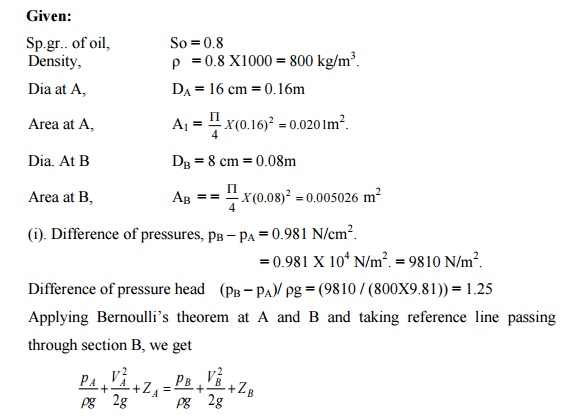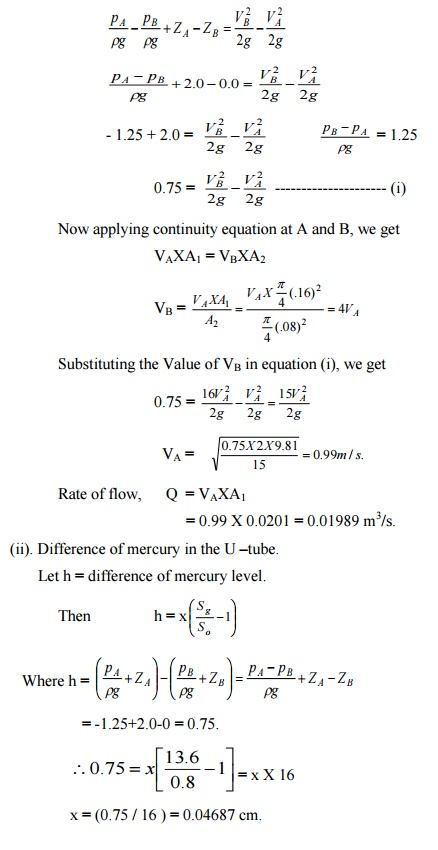Expression for loss of head due to friction in pipes or Darcy -Weisbach Equation.

Consider a uniform horizontal pipe, having steady flow as shown figure. Let 1 -1 and 2-2 is two sections of pipe.

Let P1 = pressure intensity at section 1-1. Let P2 = Velocity of flow at section 1-1.

L = length of the pipe between the section 1-1 and 2-2 d = diameter off pipe.

f1 = Frictional resistance per unit wetted area per unit velocity. hf = loss of head due to friction.

And P2,V2 = are the values of pressure intensity and velocity at section 2-2.

Applying   Bernoulli’s – 1 equation &2-2   between   section 1.1,1.2(previous)

Total head 1-1 = total head at 2-2 + loss of head due to friction between 1-1&2-2 (P1/?g) 1 2+/2g)(V+Z1 = (P2/?g)2 2 / +2g) +(VZ2+hf ————(1)

but Z1 = Z1 [ pipe is horizontal ]

V1= V2 [ diameter of pipe is same at 1-1 & 2-2]

(1) becomes,

(P1/ ?g)2/?g)+h=f(P hf = (P1/ ?g)-(P2/?g)

frictional resistance = frictional resistance per unit wetted area per unit velocity X wetted area X velocity 2.

F = f1 x ?d l x V2 [ Wetted area = ?d x L, and Velocity V = V1 = V2] F1 = f1xPxLxV2 ———– (2). [?d = wetted perimeter = p]

The forces acting on the fluid between section 1-1 and 2-2 are,

1) Pressure force at section 1-1 = P1X A

2) Pressure force at section 2-2 = P2 X A

3). Frictional force F1

Resolving all forces in the horizontal direction.,

P1 A -P2A -F1 = 0

(P1-P2)A = F= f1xPxLxV2

(P1-P2) = (f1xPxLxV2 / A ).

But from (1) we get

P1 -P2 = ?ghhf

Equating the values of (P1 -P2) we get

?ghf   = h(f1xPxLxV2 / A ).

hf = (f1  /   ?g)   X   (P/A)   X   LX   V2

(P/A) = (?d / (?d2/4)) = (4/d)

Hence, hf = ( f1 /   ?g)   x   (.   4/d)   x   LxV2

hf = 4 fLV 2 / 2gd

This equation is known as Darcy -Weisbach equation. This equation is commonly used to find loss of head due to friction in pipes.

Problem

Water flows through a pipe AB 1.2m diameter at 3 m/s and then passes through a pipe BC 1.5 m diameter at C, the pipe branches. Branch CD is 0.8m in diameter and carries one third of the flow in AB. The flow velocity in branch CE is 2.5 m/s. Find the volume rate of flow in AB, the velocity in BC, the velocity in CD and the diameter of CE.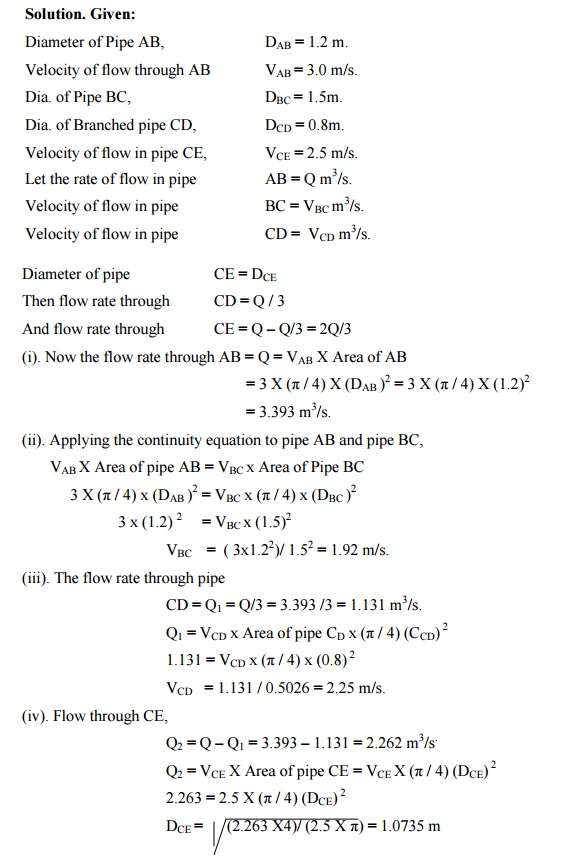1.       What is meant by energy loss in a pipe?

When the fluid flows through a pipe, it loses some energy or head due to frictional     resistance and other reasons. It is called energy loss. The losses are classified as; Majorlosses and Minor losses

2.       Explain the major losses in a pipe.

The major energy losses in a pipe is mainly due to the frictional resistance caused by           the shear force between the fluid particles and boundary walls of the pipe and also due to                viscosity of the fluid.

3.       Explain minor losses in a pipe.

The  loss  of  energy  or  head  due  to  change  of  velocity  of  the  flowing  fluid  in             magnitude  or direction is called minor losses. It includes: sudden expansion of the pipe,                   sudden contraction of the pipe, bend in a pipe, pipe fittings and obstruction in the pipe, etc.

4.       State Darcy-Weisbach equation OR What is the expression for head loss due to friction?

hf       = 4flv2   / 2gd

where, h f = Head loss due to friction (m), L = Length of the pipe (m),

d = Diameter of the pipe (m), V = Velocity of flow (m/sec)

f = Coefficient of friction where V varies from 1.5 to 2.0.

5. What are the factors influencing the frictional loss in pipe flow? Frictional resistance for the turbulent flow is,

n

i. Proportional to v

ii. Proportional to the density of fluid. iiiProportional to the area of surface in contact. iv. Independent of pressure.

v. Depend on the nature of the surface in contact.

6. What is compound pipe or pipes in series?

When the pipes of different length and different diameters are connected end to end, then the pipes are called as compound pipes or pipes in series.

7. What is mean by parallel pipe and write the governing equations.

When the pipe divides into two or more branches and again join together downstream to form a single pipe then it is called as pipes in parallel. The governing equations are:

Q1 = Q2 + Q3 hf1 = hf2

8. Define equivalent pipe and write the equation to obtain equivalent pipe diameter.

The single pipe replacing the compound pipe with same diameter without change in discharge and head loss is known as equivalent pipe.

9. What is meant by Moody‘s chart and what are the uses of Moody‘s chart?

The basic chart plotted against Darcy-Weisbach friction factor against Reynold’s Number (Re) for the variety of relative roughness and flow regimes. The relative roughness is the ratio of the mean height of roughness of the pipe and its diameter (?/D).

Moody‘s  diagram  is  accurate  to  about  15%  for  design  calculations  and  used  for  a

large number of applications. It can be used for non-circular conduits and also for open channels.

10. Define the terms a) Hydraulic gradient line [HGL] b) Total Energy line [TEL]

Hydraulic gradient line: It is defined as the line which gives the sum of pressure head and datum head of a flowing fluid in a pipe with respect the reference line.

HGL = Sum of Pressure Head and Datum head

Total energy line: Total energy line is defined as the line which gives the sum of pressure head, datum head and kinetic head of a flowing fluid in a pipe with respect to some reference line.

TEL = Sum of Pressure Head, Datum head and Velocity head

11. What do you understand by the terms a) major energy losses , b) minor energy losses Major energy losses : –

This loss due to friction and it is calculated by Darcy weis bach formula and chezys formula .

Minor energy losses :- This is due to

i.                   Sudden expansion in pipe .

ii.                 Sudden contraction in pipe .

iii.              Bend in pipe .

iv.              Due to obstruction in pipe .

12 .  Give an expression for loss of head due to sudden enlargement of the pipe :- he =

(V1-V2)2 /2g

Where              he = Loss of head due to sudden enlargement of pipe .

V1 = Velocity of flow at section 1-1

V2 = Velocity of flow at section 2-2

13.  Give an expression for loss of head due to sudden contraction : –

hc =0.5 V2/2g

Where              hc = Loss of head due to sudden contraction .

V = Velocity at outlet of pipe.

14. Give an expression for loss of head at the entrance of the pipe : – hi =0.5V2/2g

where  hi = Loss of head at entrance of pipe .

15.                    What are the basic educations to solve the problems in flow through branched pipes?

i. Continuity equation .

ii.  Bernoullis formula .

iii. Darcy weisbach equation .

16.                   Mention the general characteristics of laminar flow.

�         There is a shear stress between fluid layers

�         No slip’ at the boundary

�         The flow is rotational

There is a continuous dissipation of energy due to viscous shear

Hydraulic gradient line: It is defined as the line which gives the sum of pressure head ( P/?g) and datum head (z) of a flowing fluid in a pipe with respect to some reference line or is the line which is obtained by joining the top of all vertical ordinates, showing the pressure head ( P/?g) of a pipe from the center of the pipe.

Boundary Layer

Introduction

It is defined as the line which gives the sum of pressure head ( P/?g) and datum head (z) of a flowing fluid in a pipe with respect to some reference line or is the line which is obtained by joining the top of all vertical ordinates, showing the pressure head ( P/?g) of a pipe from the center of the pipe. It is briefly written as H.G.L

Major energy loss and minor energy loss in pipe

The loss of head or energy due to friction in pipe is known as major loss while the loss of energy due to change of velocity of the flowing fluid in magnitude or direction is called minor loss of energy.

Total Energy line

It is defined as the line, which gives sum of pressure head, datum head and kinetic head of a flowing fluid in a pipe with respect to some reference line.

Equivalent pipeline

An Equivalent pipe is defined as the pipe of uniform diameter having loss of head and discharge of a compound pipe consisting of several pipes of different lengths and diameters.

Water Hammer in pipes.

In a long pipe, when the flowing water is suddenly brought to rest by closing the valve or by any similar cause, there will be a sudden rise in pressure due to the momentum of water being destroyed. A pressure wave is transmitted along the pipe. A sudden rise in pressure has the effect of hammering action on the walls of the pipe. This phenomenon of rise in pressure is known as water hammer or hammer blow.

The boundary layer is called laminar, if the Renolds number of the flow is defined as Re = U x X / v is less than 3X105

If the Renolds number is more than 5X105, the boundary layer is called turbulent boundary.

Where, U = Free stream velocity of flow X = Distance from leading edge v = Kinematic viscosity of fluid

Chezy’s formula.

Chezy’s formula is generally used for the flow through open channel.

V =C.Rt(mi)

Where , C = chezy’s constant, m = hydraulic mean depth and i = hf/ L.

Problem 1

A crude of oil of kinematic viscosity of 0.4 stoke is flowing through a pipe of diameter 300mm at the rate of 300 litres/sec. find the head lost due to friction for a length of 50m of the pipe.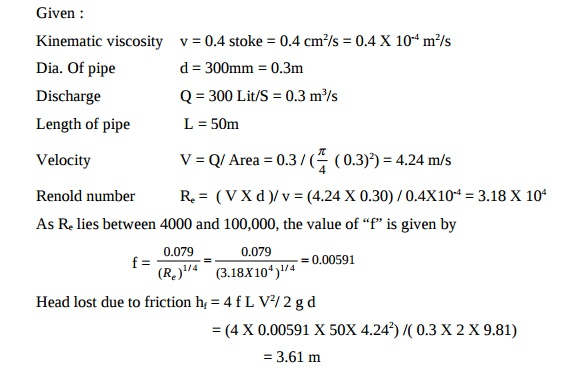Problem 2

Find the type of flow of an oil of relative density 0.9 and dynamic viscosity 20 poise, flowing through a pipe of diameter 20 cm and giving a discharge of 10 lps. Solution :

s = relative density = Specific gravity = 0.9 m = Dynamic viscosity = 20 poise = 2 Ns/m2.

Dia of pipe D = 0.2 m; Discharge Q = 10 lps = (10 / 1000) m3/s; Q = AV. So V = Q / A = [10 / (1000 X ( p4 ( 0.2)2) )] = 0.3183 m/s.

Kinematic viscosity = v = m / ? = [ 2 / (0.9X1000)] = 2.222X10-3 m2 / s. Reynolds number Re = VD / v

Re = [0.3183 X 0.2 / 2.222X10-3] = 28.647; Since Re ( 28.647) < 2000,

It is Laminar flow.

Formula for finding the loss of head due to entrance of pipe hi

hi  = 0.5 ( V2 / 2g)

Formula to find the Efficiency of power transmission through pipes

n = ( H – hf) / H

where, H = total head at inlet of pipe. hf = head lost due to friction

Problem 3

Hydro dynamically smooth pipe carries water at the rate of 300 lit/s at 20oC (r = 1000 kg/m3n = 10-6 m2/s) with a head loss of 3m in 100m length of pipe.

Determine the pipe diameter. Use f = 0.0032 + (0.221)/ (Re)0.237 equation for f where hf = ( fXLXV2)/ 2gd and Re = (rVD/m)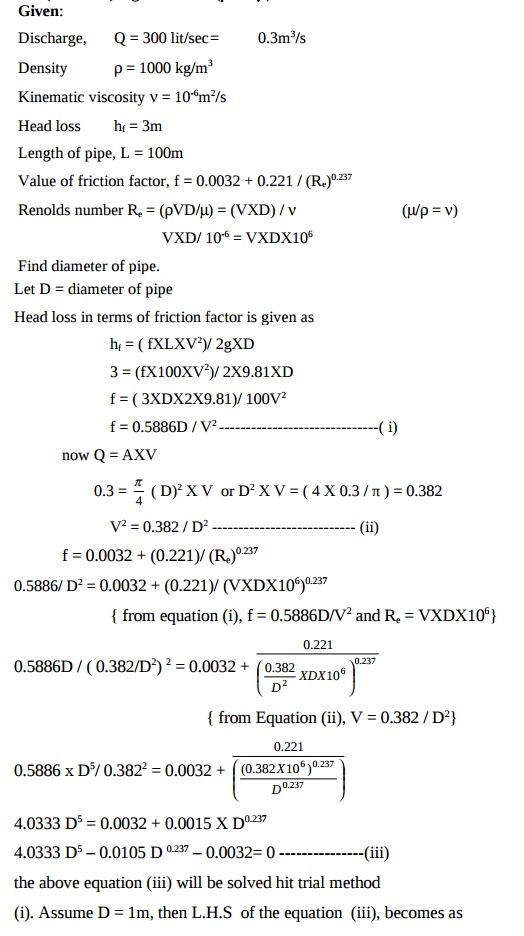(i) Convergent flows: Negative pressure gradients

If flow over a boundary occurs when there is a pressure decrease in the direction of flow, the fluid will accelerate and the boundary layer will become thinner.

This is the case for convergent flows.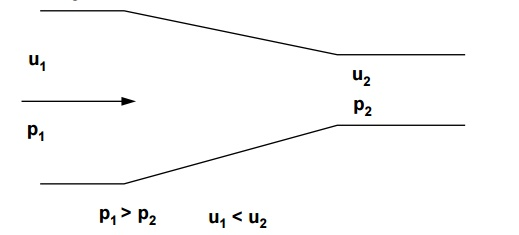The accelerating fluid maintains the fluid close to the wall in motion. Hence the flow remains stable and turbulence reduces. Boundary layer separation does not occur.

(ii) Divergent flows: Positive pressure gradients

When the pressure increases in the direction of flow the situation is very different. Fluid outside theboundary layer has enough momentum to overcome this pressure which is trying to push it backwards. The fluid within the boundary layer has so little momentum that it will very quickly be brought to rest,and possibly reversed in direction. If this reversal occurs it lifts the boundary layer away from the surfaceas shown below.This phenomenon is known as  boundary layer separation.

At the edge of the separated boundary layer, where the velocities change direction, a line of vortices occur (known as a vortex sheet). This happens because fluid to either side is moving in the opposite direction.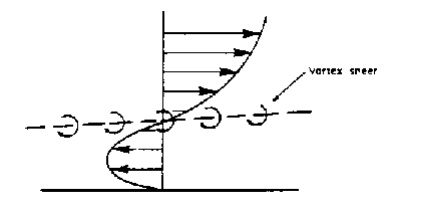This boundary layer separation and increase in the turbulence because of the vortices results in very large energy losses in the flow.

These separating / divergent flows are inherently unstable and far more energy is lost than in parallel or convergent flow.

(iii) A divergent duct or diffuser

The increasing area of flow causes a velocity drop (according to continuity) and hence a pressure rise(according to the Bernoulli equation).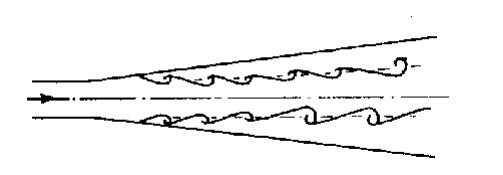Increasing the angle of the diffuser increases the probability of boundary layer separation. In a Venturi meter it has been found that an angle of about 6 o provides the optimum balance between length of meter and danger of boundary layer separation which would cause unacceptable pressure energy losses.

(iv) Tee-Junctions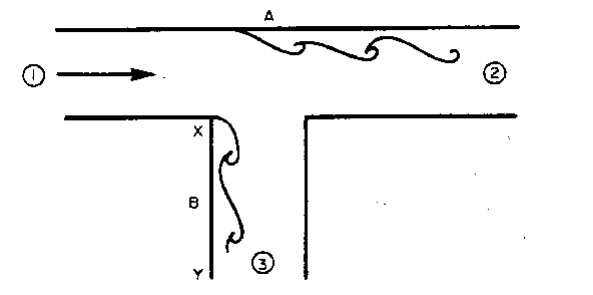Assuming equal sized pipes, as fluid is removed, the velocities at 2 and 3 are smaller than at 1, the entrance to the tee. Thus the pressure at 2 and 3 are higher than at 1. These two adverse pressure gradients can cause the two separations shown in the diagram above.

(v) Y-Junctions

Tee junctions are special cases of the Y-junction with similar separation zones occurring. See the diagram below.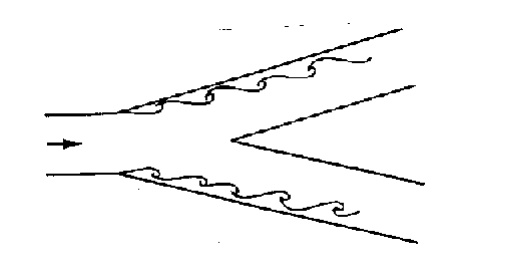Downstream, away from the junction, the boundary layer reattaches and normal flow occurs i.e. the effect of the boundary layer separation is only local. Nevertheless fluid downstream of the junction will have lost energy.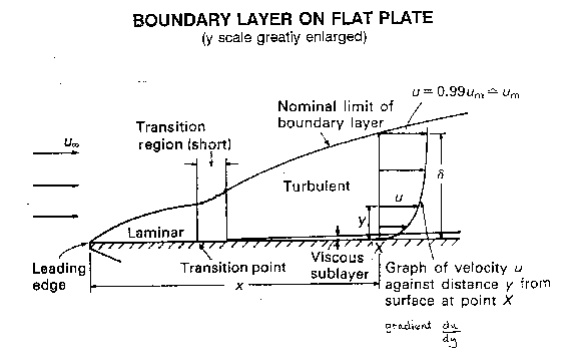When a fluid flows over a stationary surface, e.g. the bed of a river, or the wall of a pipe, the fluidtouching the surface is brought to rest by the shear stress Ro at the wall. The velocity increases from thewall to a maximum in the main stream of the flow.

Boundary Layers

When a fluid flows over a stationary surface, e.g. the bed of a river, or the wall of a pipe, the fluidtouching the surface is brought to rest by the shear stress  to  at the wall. The velocity increases from thewall to a maximum in the main stream of the flow.Looking at this two-dimensionally we get the above velocity profile from the wall to the centre of the flow.

This profile doesn’t just exit, it must build up gradually from the point where the fluid starts to flow past the surface – e.g. when it enters a pipe.

If we consider a flat plate in the middle of a fluid, we will look at the build up of the velocity profile as the fluid moves over the plate.

Upstream the velocity profile is uniform, (free stream flow) a long way downstream we have the velocity profile we have talked about above. This is the known as fully developed flow. But how do we get to that state?

This region, where there is a velocity profile in the flow due to the shear stress at the wall, we call the boundary layer. The stages of the formation of the boundary layer are shown in the figure below:We define the thickness of this boundary layer as the distance from the wall to the point where the velocity is 99% of the ‘free stream’ velocity, the velocity in the middle of the pipe or river.

boundary layer thickness, d = distance from wall to point where u = 0.99 umainstream

The value of d will increase with distance from the point where the fluid first starts to pass over the boundary – the flat plate in our example. It increases to a maximum in fully developed flow.

Correspondingly, the drag force D on the fluid due to shear stress to at the wall increases from zero at the start of the plate to a maximum in the fully developed flow region where it remains constant. We can calculate the magnitude of the drag force by using the momentum equation. But this complex and not necessary for this course.

Our interest in the boundary layer is that its presence greatly affects the flow through or round an object. So here we will examine some of the phenomena associated with the boundary layer and discuss why these occur.

Previously we noted that the boundary layer grows from zero when a fluid starts to flow over a solid surface. As is passes over a greater length more fluid is slowed by friction between the fluid layers close to the boundary. Hence the thickness of the slower layer increases.

The fluid near the top of the boundary layer is dragging the fluid nearer to the solid surface along. The mechanism for this dragging may be one of two types:

The first type occurs when the normal viscous forces (the forces which hold the fluid together) are large enough to exert drag effects on the slower moving fluid close to the solid boundary. If the boundary layer is thin then the velocity gradient normal to the surface, (du/dy), is large so by Newton’s law of viscosity the shear stress, = m (du/dy), is also large. The corresponding force may then be large enough to exert drag on the fluid close to the surface.

As the boundary layer thickness becomes greater, so the velocity gradient become smaller and the shear stress decreases until it is no longer enough to drag the slow fluid near the surface along. If this viscous force was the only action then the fluid would come to a rest.

It, of course, does not come to rest but the second mechanism comes into play. Up to this point the flow has been laminar and Newton’s law of viscosity has applied. This part of the boundary layer is known as the laminar boundary layer

The viscous shear stresses have held the fluid particles in a constant motion within layers. They become small as the boundary layer increases in thickness and the velocity gradient gets smaller. Eventually they are no longer able to hold the flow in layers and the fluid starts to rotate.This causes the fluid motion to rapidly becomes turbulent. Fluid from the fast moving region moves to the slower zone transferring momentum and thus maintaining the fluid by the wall in motion. Conversely, slow moving fluid moves to the faster moving region slowing it down. The net effect is an increase in momentum in the boundary layer. We call the part of the boundary layer the turbulent boundary layer.

At points very close to the boundary the velocity gradients become very large and the velocity gradients become very large with the viscous shear forces again becoming large enough to maintain the fluid in laminar motion. This region is known as the laminar sub-layer. This layer occurs within the turbulent zone and is next to the wall and very thin – a few hundredths of a mm.

Surface roughness effect

Despite its thinness, the laminar sub-layer can play a vital role in the friction characteristics of the surface.

This is particularly relevant when defining pipe friction – as will be seen in more detail in the level 2 module. In turbulent flow if the height of the roughness of a pipe is greater than the thickness of the laminar sub-layer then this increases the amount of turbulence and energy losses in the flow. If the height of roughness is less than the thickness of the laminar sub-layer the pipe is said to be smooth and it has little effect on the boundary layer.

In laminar flow the height of roughness has very little effect

Boundary layers in pipes

As flow enters a pipe the boundary layer will initially be of the laminar form. This will change depending on the ration of inertial and viscous forces; i.e. whether we have laminar (viscous forces high) or turbulent flow (inertial forces high).

From earlier we saw how we could calculate whether a particular flow in a pipe is laminar or turbulent using the Reynolds number.

Re = rud / m

(r = density   u = velocity   m = viscosity  d = pipe diameter)

Laminar flow:                          Re < 2000

Transitional flow: 2000 < Re < 4000

Turbulent flow:                        Re > 4000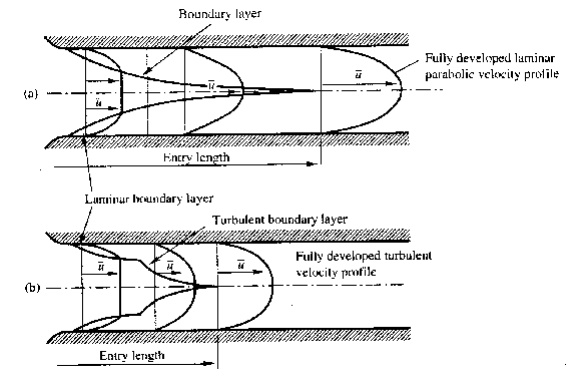If we only have laminar flow the profile is parabolic – as proved in earlier lectures – as only the first part of the boundary layer growth diagram is used. So we get the top diagram in the above figure.

If turbulent (or transitional), both the laminar and the turbulent (transitional) zones of the boundary layer growth diagram are used. The growth of the velocity profile is thus like the bottom diagram in the above figure.

Once the boundary layer has reached the centre of the pipe the flow is said to be fully developed. (Note that at this point the whole of the fluid is now affected by the boundary friction.)

The length of pipe before fully developed flow is achieved is different for the two types of flow. The length is known as the entry length.

Laminar flow entry length   120  diameter

Turbulent flow entry length   60  diameter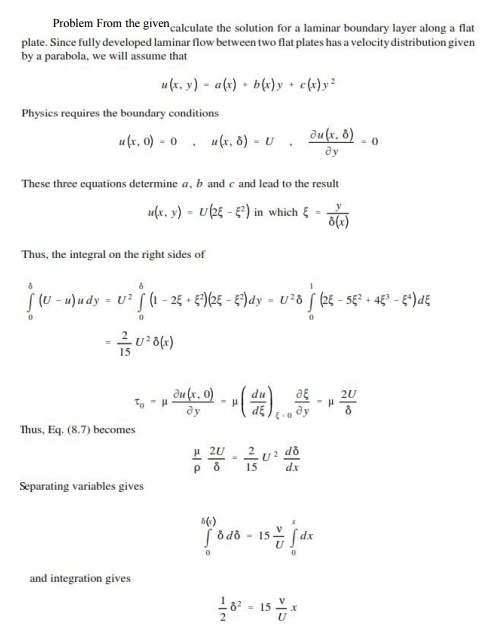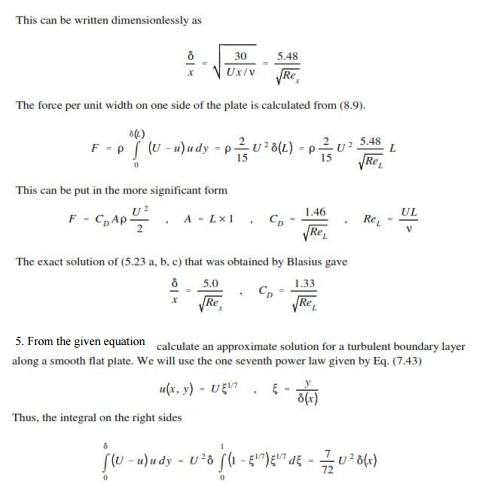BOUNDARY LAYER

1. Mention the range of Reynold’s number for laminar and turbulent flow in a pipe.

If the Reynolds number is less than 2000, the flow is laminar. But if the Reynold’s number is greater than 4000, the flow is turbulent flow.

2. What does Haigen-Poiseulle equation refer to?

The equation refers to the value of loss of head in a pipe of length L due to viscosity in a laminar flow.

3. What are the factors to be determined when viscous fluid flows through the circular pipe? The factors to be determined are:

i. Velocity distribution across the section.

ii. Ratio of maximum velocity to the average velocity. iii. Shear stress distribution.

iv. Drop of pressure for a given length

4. Define kinetic energy correction factor?

Kinetic energy factor is defined as the ratio of the kinetic energy of the flow per sec based on  actual velocity across a section to the kinetic energy of the flow per sec based on average  velocity across the same section. It is denoted by (?).

K.  E  factor  (?)  =  K.E  per  sec  based  on  actual  velocity  /  K.E  per  sec  based  on  Average velocity

5. Define momentum correction factor (?):

It is defined as the ratio of momentum of the flow per sec based on actual velocity to the  momentum of the flow per sec based on average velocity across the section.

?= Momentum  per  sec  based  on  actual  velocity/Momentum  Per  sec  based  on  average  velocity

6. Define Boundary layer.

When  a real  fluid  flow passed a solid  boundary,  fluid  layer is  adhered  to  the solid  boundary. Due to adhesion fluid undergoes retardation thereby developing a small region in the  immediate vicinity of the boundary. This region is known as boundary layer.

7. What is mean by boundary layer growth?

At  subsequent  points  downstream  of  the  leading  edge,  the  boundary  layer  region  increases because the retarded fluid is further retarded. This is referred as growth of boundary  layer.

8. Classification of boundary layer.

(i) Laminar boundary layer,

(ii) Transition zone,

(iii)Turbulent boundary layer.

9. Define Laminar boundary layer.

Near the leading edge of the surface of the plate the thickness of boundary layer is small  and flow is laminar. This layer of fluid is said to be laminar boundary layer.

The length of the plate from the leading edge, upto which laminar boundary layer exists  is called as laminar zone. In this zone the velocity profile is parabolic.

10. Define transition zone.

After laminar zone, the laminar boundary layer becomes unstable and the fluid motion  transformed to turbulent boundary layer. This short length over which the changes taking place

is called as transition zone. 11. Define Turbulent boundary.

Further downstream of transition zone, the boundary layer is turbulent and continuous to grow in thickness. This layer of boundary is called turbulent boundary layer.

12. Define Laminar sub Layer

In the turbulent boundary layer zone, adjacent to the solid surface of the plate the velocity variation is influenced by viscous effects. Due to very small thickness, the velocity distribution is almost linear. This region is known as laminar sub layer.

13. Define Boundary layer Thickness.

It is defined as the distance from the solid boundary measured in y-direction to the point, where the velocity of fluid is approximately equal to 0.99 times the free stream velocity

(U) of the fluid. It is denoted by ?.

14.List the various types of boundary layer thickness.

Displacement thickness(?*), Momentum thickness(?), Energy thickness(?**)

15. Define displacement thickness.

The displacement thickness (?) is defined as the distance by which the boundary should be displaced to compensate for the reduction in flow rate on account of boundary

layer formation.

16. Define momentum thickness.

The momentum thickness (?) is defined as should be displaced to compensate for the reduction account of boundary layer formation. the distance by which the boundary in momentum of the flowing fluid on

ppppppppp

17. Define energy thickness

The energy thickness (?**) is defined as the distance by which the boundary should be displaced to compensate for the reduction in kinetic energy of the flowing fluid on account of boundary layer formation.

Dimensional analysis is defined as a mathematical technique used in research work for design and conducting model tests.

Dimensional Analysis And Model Studies

INTRODUCTION

Dimensional analysis.

Dimensional analysis is defined as a mathematical technique used in research work for design and conducting model tests.

Fundamental dimensions

The fundamental units quantities such as length (L), mass (M), and time (T) are fixed dimensions known as fundamental dimensions.

Units.

Unit is defined as a yardstick to measure physical quantities like distance, area, volume, mass etc.

Derive the dimensions for velocity.

Velocity is the distance (L) travelled per unit time (T)

Velocity = Distance/ Time = [L/T] = LT-1.

Model

Model is nothing but small-scale repetition of the actual structure or machine.

List out the advantages of model analysis.

The advantages of model analysis are:

1.   The performance of hydraulic structure or machine can be easily predicted in advance from its model.

The merits of alternative design can be predicted with the help of model testing and the most economical and safe design may be finally adopted.

The three types of similarities are, 1. Geometric similarity 2. Kinematic similarity 3. Dynamic similarity

Similitude

The three types of similarities are,

1.   Geometric similarity

2.   Kinematic similarity

3.   Dynamic similarity

Scale ratio.

Scale ratio is the ratio of linear dimension in the model and prototype which are equal in a geometric similarity. It is denoted by Lr.

Lr = Lp/Lm = bp/bm = Dp/Dm

Dynamic similarity.

It means the similarity of forces at corresponding points in the model and prototype is equal.

Types of forces in a moving fluid.

The types of forces in a moving fluid are,

1.   Inertia force (fi)

2.   Viscous force (fv)

3.   Gravity force (Fg)

4.   Pressure force (Fp)

5.   surface tension (Fs)

6.   Elastic force (Fe)

Dimensionless numbers.

Dimensionless numbers are the numbers obtained by dividing inertia force or gravity force or pressure force or elastic force or surface tension. They are called as non-dimensional parameters.

Surface tension.

Surface tension force is defined as the product of surface tension and length of surface of flowing fluid.

Pressure force.

Pressure force is the product of pressure intensity and cross-sectional area of the flowing fluid in case of pipe flow.

Elastic force.

Elastic force is defined as the product of elastic stress and the area of flowing fluid.

Types of dimensionless numbers.

The types of dimensionless numbers are:

1.   Reynold’s number

2.   Froude’s number

3.   Euler’s number

4.   Weber’s number

5.   Mach’s number

Reynold’s number.

Reynold’s number is defined as the ratio of inertia force of flowing fluid and viscous force of the fluid. It is denoted by (Re)

Re = V x d / v = ? V d / ?

Froude’s number

Froude’s number is defined as square root of ratio of inertia force of flowing fluid to gravity. It is denoted as Fe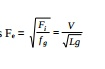The classifications of models are i. Undistorted models ii. Distorted models

Classification of models

The classifications of models are

i.             Undistorted models

ii.    Distorted models

Undistorted model

If the scale ratio for the linear dimensions of the model and prototype is same, then the model is said to be undistorted model.

Distorted model

A distorted model is said to be a distorted model only when it is not geometrically similar to prototype.

Advantages of distorted models.

The advantages of distorted models are,

1.   The vertical dimensions of the model can be measured accurately.

2.   The cost of model can be reduced.

3.   Turbulent flow in the model can be maintained.

The types of model laws are 1. Reynolds’s model laws 2. Froude’s model laws 3. Euler’s model laws 4. Weber’s model laws 5. Mach’s model laws

Types of model laws

The types of model laws are

1.   Reynolds’s model laws

2.   Froude’s model laws

3.   Euler’s model laws

4.   Weber’s model laws

5.   Mach’s model laws

Application of Froude’s model laws

Froude’s model laws is applied in

1.Free surface flow such as flow over spillways, weirs, sluices, channels etc

2.Flow of jet from an orifice or nozzle

3.Where waves are likely to be formed on surface

4.Where fluids of different densities flow over one anther

Weber’s model laws

When surface tensile forces alone are predominant a model may be taken to be dynamically similar to the prototype when ratio of inertial to the surface tensile forces is the same in the model and prototype.

(We) model = (We) prototype

The Buckingham’s ‘ Pi ‘ theorem is a key theorem in dimensional analysis. The theorem states that if we have a physically meaningful equation involving a certain number, n of physical variables and these variables are expressible in terms of k independent fundamental physical qualities, then the original expression is equivalent to an equation involving a set of p = n – k dimensionless variables constructed from the original variables. For the purpose of the experimenter, different systems which share the same description in terms of these dimensionless numbers are equivalent.

In mathematical terms, if we have a physically meaningful equation such as f ( q1, q2,��..qn ) = 0

where the qi are the n physical variables and they are expressed in terms of k independent physical units, the above equation can be restated as

F (?1, ?2���. ?p) = 0

Where, the ?i are the dimensionless parameters constructed from the qi by p = n-k equations of the form

?i  = q1m, q2,m��..qnm

Where the exponents mi are the rational numbers. The use of the ?i the dimensionless parameters was introduced by Edge Buckingham in his original 1914 paper on the subject from which the theorem draws its name.

Most importantly, the Buckingham ? theorem provides a method for computing sets of dimensionless parameters is not unique: Buckingham’s theorem only provides a way of generating sets of dimensionless parameters and will not choose the most ‘physically meaningful’.

Proofs of the ? theorem often begin by considering the space of fundamental and derived physical unit’s vector space, with the fundamental units as basis vectors and with multiplication of physical units as the ‘Vector addition’ operation and raising to powers as the ‘scalar multiplication’ operation.

Making the physical units match across sets of physical equations can then the regarded as imposing linear constraints in the physical units vector space.

The theorem describes how every physical meaningful equation involving variables can be equivalently rewritten as an equation of n – m dimensionless parameters, where m is the number of fundamental units used. Furthermore and most importantly it provides a method for computing these dimensionless parameters from the given variables, even if the form of the equation is still unknown.

Two systems for which these parameters coincide are called similar; they are equivalent for the purposes of the experimental list who wants to determine the form of the equation can choose the most convenient one.

The ? theorem uses linear algebra: the space of all possible physical units can be seen as a vector space over the rational numbers if we represent a unit as the set of exponents needed for the fundamental units ( with a power of zero if the particular fundamental unit is not present) Multiplication of physical units is then represented by vector addition within this vector space. The algorithm of the ?

theorem is essentially a Gauss – Jordan elimination carried out in this vector space.

Problem 1

The resisting force of (R) of a supersonic flight can be considered as dependent upon the length of aircraft ‘l’, velocity ‘V’, air viscosity ‘?’, air density ‘?’, and bulk modulus of air ‘k’. Express the functional relationship between these variables and the resisting force.

Solution:

Step 1. R = f ( l, V, ?, ?, k ) ? ( R, l, V, ?, ?, k) = 0

Number of variables, n = 6 Number of primary variable, m = 3

Number of ? terms = n – m = 6 – 3 = 3 f (?1, ?2, ?3) = 0

Step 2.  Assume l, V and ? to be the repeating variables.

?1  = lx, Vy, ?z,R

MOLOTO = [ L]X [ LT-1y [ML-3]z [MLT-2]Problem 2

A Ship is 300m long moves in seawater, whose density is 1030 kg/m3, A 1:100 model of this to be tested in a wind tunnel. The velocity of air in the wind tunnel around the model is 30m/s and the resistance of the model is 60N. Determine the velocity of ship in seawater and also the resistance of the ship in sea water. The density of air is given as 1.24 kg/m3. Take the Kinematic viscosity of seawater and air as 0.012 stokes and 0.018 stokes respectively.

Given:

For prototype,

Length                                                                          Lp = 300m

Fluid                                                                              = Sea water

Density of water                                                           = 1030 kg/m3Problem 3

A 7.2 m height and 15m long spill way discharges 94 m3/s discharge under a head of 2.0m. If a 1:9 scale model of this spillway is to be constructed, determine model dimensions, head over spillway model and the model discharge. If model experience a force of 7500N (764.53Kgf), determine force on the prototype.

Given:

For prototype: height  hp = 7.2m

Length,                         Lp = 15m

Discharge                    Qp = 94 m3/s

Head,                                                                            Hp = 2.0m

Size of model = 1/9. of the size of prototype

Linear scale ratio,        Lr = 9

Force experienced by model  Fp = 7500N

Find : (i) Model dimensions i.e., height and length of model (hm and Lm) (ii). Head over model i.e., Hm

(iii). Discharge through model i.e., Qm (iv). Force on prototype (i.e., Fp)

(i). Model dimensions ( hm and Lm )

hp/ hm  = Lp/ Lm = Lr = 9

hm = hp / 9 = 7.2 / 9 = 0.8 m Lm = Lp / 9 = 15 / 9 = 1.67 m.

(ii). Head over model (Hm)

hp/ Hm = Lr = 9

Hm = Hp / 9 = 2/9 = 0.222 m. (iii). Discharge through model (Qm)

Using equation we get, Qp / Qm = Lr2.5

Qm = (Qp / Lr2.5) = 94 / 92.5 = 0.387 m3/s. (iv). Force on the prototype(Fp)

Using Fr = Fp / Fm = Lr3

Fp = Fm X Lr3 7500X93 = 5467500N.

Problem 4

A quarter scale turbine model is tested under a head of 12m. The full-scale turbine is to work under a head of 30m and to run at 428 rpm. Find N for model. If model develops 100 kW and uses 1100 l/s at this speed, what power will be obtained from full scale turbine assuming its n is 3% better than that of model.

Solution :

Dm / Dp = 1/4 ; Hm = 30 m; Np = 428 rpm. w.k.t.DIMENSIONAL ANALYSIS AND MODEL STUDIES

1. Define dimensional analysis.

Dimensional analysis is a mathematical technique which makes use of the study of dimensions as an aid to solution of several engineering problems. It plays an important role in research work.

2. Write the uses of dimension analysis?

�    It helps in testing the dimensional homogeneity of any equation of fluid motion.

� It helps in deriving equations expressed in terms of non-dimensional parameters.

� It helps in planning model tests and presenting experimental results in a systematic manner.

3. Define dimensional homogeneity.

An equation is said to be dimensionally homogeneous if the dimensions of the terms on its LHS are same as the dimensions of the terms on its RHS.

4. Mention the methods available for dimensional analysis. Rayleigh method,

Buckinghum ? method

5. State Buckingham? theorem.

It states that if there are ‘n’ variables (both independent & dependent variables) in a physical phenomenon and if these variables contain m functional dimensions and are related by a dimensionally homogeneous equation, then the variables are arranged into n-m dimensionless terms. Each term is called ? term.

6. List the repeating variables used in Buckingham ? theorem. Geometrical Properties  l, d, H, h, etc, Flow Properties  v, a, g, ?, Q, etc,

Fluid Properties – ?, ??, etc.

7. Define model and prototype.

The small scale replica of an actual structure or the machine is known as its Model, while the actual structure or machine is called as its Prototype. Mostly models are much smaller than the corresponding prototype.

8. Write the advantages of model analysis.

� Model test are quite economical and convenient.

� Alterations can be continued until most suitable design is obtained.

� Modification of prototype based on the model results.

� The information about the performance of prototype can be obtained well in advance.

9. List the types of similarities or similitude used in model anlaysis.

Geometric similarities, Kinematic similarities, Dynamic similarities 10. Define geometric similarities

It exists between the model and prototype if the ratio of corresponding lengths, dimensions in the model and the prototype are equal. Such a ratio is known as Scale Ratio.

11. Define kinematic similarities

It exists between the model and prototype if the paths of the homogeneous moving particles are geometrically similar and if the ratio of the flow properties is equal.

12. Define dynamic similarities

It exits between model and the prototype which are geometrically and kinematic similar and if the ratio of all forces acting on the model and prototype are equal.

13.                                     Mention the various forces considered in fluid flow. Inertia force,

Viscous force, Gravity force, Pressure force,

Surface Tension force, Elasticity force

14.Define model law or similarity law.

The condition for existence of completely dynamic similarity between a model and its prototype are denoted by equation obtained from dimensionless numbers. The laws on which the models are designed for dynamic similarity are called Model laws or Laws of Similarity.

15.                                     List the various model laws applied in model analysis. Reynolds Model Law,

Froudes Model Law, Euler’s Model Law, Weber Model Law, Mach Model Law

16.State Euler’s model law

In a fluid system where supplied pressures are the controlling forces in addition to inertia forces and other forces are either entirely absent or in-significant the Eulers number for both the model and prototype which known as Euler Model Law.

17. State Webers model law

When surface tension effect predominates in addition to inertia force then the dynamic similarity is obtained by equating the Webers number for both model and its prototype, which is called as Weber Model Law.

18. State Mach’s model law

If in any phenomenon only the forces resulting from elastic compression are significant in addition to inertia forces and all other forces may be neglected, then the dynamic similarity between model and its prototype may be achieved by equating the Machs number for both the systems. This is known Mach Model Law.

19. Classify the hydraulic models.

The hydraulic models are classified as: Undistorted model & Distorted model 20. Define undistorted model

An undistorted model is that which is geometrically similar to its prototype, i.e. the scale ratio for corresponding linear dimensions of the model and its prototype are same.

21. Define distorted model

Distorted models are those in which one or more terms of the model are not identical with their counterparts in the prototype.

22. Define Scale effect

An effect in fluid flow that results from changing the scale, but not the shape, of a body around which the flow passes.

Rigid body motion theory is a fundamental and well-established part of physics. It is based on the approximation that for stiff materials, any force applied to a body produces a negligible deformation. Thus, the only change a force can produce is change in the center of mass motion and change in the rotational motion. This means that simulation of even complex bodies is relatively simple, and thus this method has become popular in the computer simulation field.

Given the forces acting on the body, the motion can be determined using ?? ??for translational motion, and a similar relation for rotational motion .

The rigid body motion model has traditionally been applied in range analysis in CAD and for computer animation where deformation is not required. If the deformation is not negligible, then the approximation does not hold, and we need to start over and come up with some other model. There exists many different models, but the two models which have emerged to become the most widely used in practice are: mass-spring models and statics models solved using the Finite Element Method (FEM).

Mass-spring models represent bodies as discrete mass-elements, and the forces between them are transmitted using explicit spring connections (‘spring’ is a historical term, and is not limited to pure Hooke interactions). Given the forces acting on an element, we can determine its motion using . The motion of the entire body is then implicitly described by the motion of its elements.

Mass-spring models have traditionally been applied mostly for cloth simulation. Statics models are based on equilibrium relations, and thus make the approximation that the effect of dynamics are negligible. Relations between the strain and stress fields of a body are set up, and through specifying known values of these fields, through for example specifying forces acting on the body, the unknown parts can be determined. These relations form differential equations, and the known values are boundary values. The FEM is an effective method for solving boundary value problems, and has thus given its name to these types of problems. Statics models have traditionally been applied in stress and displacement analysis systems in CAD.

Strength The ability to sustain load.

�        Stiffness Push per move; the ratio of deformation to associated load level.

�        Stability The ability of a structure to maintain position and geometry. Instability involves collapse that is not initiated by material failure. External stability concerns the ability of a structure’s supports to keep the structure in place; internal stability concerns a structure’s ability to maintain its shape.

�        Ductility The amount of inelastic deformation before failure, often expressed relative to the amount of elastic deformation.

Strength Material strength is measured by a stress level at which there is a permanent and significant change in the material’s load carrying ability. For example, the yield stress, or the ultimate stress.

Stiffness Material stiffness is most commonly expressed in terms of the modulus of elasticity: the ratio of stress to strain in the linear elastic range of material behavior.

Stability As it is most commonly defined, the concept of stability applies to structural elements and systems, but does not apply to materials, since instability is defined as a loss of load carrying ability that is not initiated by material failure.

Ductility Material ductility can be measured by the amount of inelastic strain before failure compared to the amount of elastic strain. It is commonly expressed as a ratio of the maximum strain at failure divided by the yield strain.

A tensile test is generally conducted on a standard specimen to obtain the relationship between the stress and the strain which is an important characteristic of the material.

Mechanical properties of materials

A tensile test is generally conducted on a standard specimen to obtain the relationship between the stress and the strain which is an important characteristic of the material. In the test, the uniaxial load is applied to the specimen and increased gradually. The corresponding deformations are recorded throughout the loading. Stress-strain diagrams of materials vary widely depending upon whether the material is ductile or brittle in nature. If the material undergoes a large deformation before failure, it is referred to as ductile material or else brittle material.Stress-strain diagram of a structural steel, which is a ductile material, is given.

Initial part of the loading indicates a linear relationship between stress and strain, and the deformation is completely recoverable in this region for both ductile and brittle materials. This linear relationship, i.e., stress is directly proportional to strain, is popularly known as Hooke’s law.

s = Ee

The co-efficient E is called the modulus of elasticity or Young’s modulus.

Most of the engineering structures are designed to function within their linear elastic region only.After the stress reaches a critical value, the deformation becomes irrecoverable. The corresponding stress is called the yield stress or yield strength of the material beyond which the material is said to start yielding.

In some of the ductile materials like low carbon steels, as the material reaches the yield strength it starts yielding continuously even though there is no increment in external load/stress. This flat curve in stress strain diagram is referred as perfectly plastic region.

The load required to yield the material beyond its yield strength increases appreciably and this is referred to strain hardening of the material. In other ductile materials like aluminum alloys, the strain hardening occurs immediately after the linear elastic region without perfectly elastic region.

After the stress in the specimen reaches a maximum value, called ultimate strength, upon further tretching, the diameter of the specimen starts decreasing fast due to local instability and this p henomenon is called necking.

The load required for further elongation of the material in the necking region decreases with decrease in diameter and the stress value at which the material fails is called the breaking strength. In case of brittle materials like cast iron and concrete, the material experiences smaller deformation before rupture and there is no necking.

The stress was calculated by dividing the load P by the initial cross section of the specimen. But it is clear that as the specimen elongates its diameter decreases and the decrease in cross section is apparent during necking phase. Hence, the actual stress which is obtained by dividing the load by the actual cross sectional area in the deformed specimen is different from that of the engineering stress that is obtained using undeformed cross sectional area as in equation 1.1 Though the difference between the true stress and the engineering stress is negligible for smaller loads, the former is always higher than the latter for larger loads.

Similarly, if the initial length of the specimen is used to calculate the strain, it is called engineering strain as obtained in equation 1.9

But some engineering applications like metal forming process involve large deformations and they require actual or true strains that are obtained using the successive recorded lengths to calculate the strain. True strain is also called as actual strain or natural strain and it plays an important role in theories of viscosity.

TYPES OF STRESSES :

Only two basic stresses exists : (1) normal stress and (2) shear shear stress. Other stresses either are similar to these basic stresses or are a combination of these e.g. bending stress is a combination tensile, compressive and shear stresses. Torsional stress, as encountered in twisting of a shaft is a shearing stress.

Let us define the normal stresses and shear stresses in the following sections.

Normal stresses : We have defined stress as force per unit area. If the stresses are normal to the areas concerned, then these are termed as normal stresses. The normal stresses are generally denoted by a Greek letter ( s )

This is also known as uniaxial state of stress, because the stresses acts only in one direction however, such a state rarely exists, therefore we have biaxial and triaxial state of stresses where either the two mutually perpendicular normal stresses acts or three mutually perpendicular normal stresses acts as shown in the figures below :

Tensile or compressive stresses :

The normal stresses can be either tensile or compressive whether the stresses acts out of the area or into the area

Bearing Stress : When one object presses against another, it is referred to a bearing stress ( They are in fact the compressive stresses ).

Shear stresses :

Let us consider now the situation, where the cross  sectional area of a block of material is subject to a distribution of forces which are parallel, rather than normal, to the area concerned. Such forces are associated with a shearing of the material, and are referred to as shear forces. The resulting force interistes are known as shear stresses.

The resulting force intensities are known as shear stresses, the mean shear stress being equal to

Where P is the total force and A the area over which it acts.

Stress is defined as the force per unit area. Thus, the formula for calculating stress is:

s= F/A

Where s denotes stress, F is load and A is the cross sectional area. The most commonly used units for stress are the SI units, or Pascals (or N/m2), although other units like psi (pounds per square inch) are sometimes used.

Forces may be applied in different directions such as:

Tensile or stretching

�                  Compressive or squashing/crushing

�                  Shear or tearing/cutting

�                  Torsional or twisting

This gives rise to numerous corresponding types of stresses and hence measure/quoted strengths. While data sheets often quote values for strength (e.g compressive strength), these values are purely uniaxial, and it should be noted that in real life several different stresses may be acting.

Tensile Strength

The tensile strength is defined as the maximum tensile load a body can withstand before failure divided by its cross sectional area. This property is also sometimes referred to Ultimate Tensile Stress or UTS.

Typically, ceramics perform poorly in tension, while metals are quite good. Fibres such as glass, Kevlar and carbon fibre are often added polymeric materials in the direction of the tensile force to reinforce or improve their tensile strength.

Compressive Strength

Compressive strength is defined as the maximum compressive load a body can bear prior to failure, divided by its cross sectional area.

Ceramics typically have good tensile strengths and are used under compression e.g. concrete.

Shear Strength

Shear strength is the maximum shear load a body can withstand before failure occurs divided by its cross sectional area.

This property is relevant to adhesives and fasteners as well as in operations like the guillotining of sheet metals.

Torsional Strength

Torsional strength is the maximum amount of torsional stress a body can withstand before it fails, divided by its cross sectional area.

This property is relevant for components such as shafts.

Yield Strength

Yield strength is defined as the stress at which a material changes from elastic deformation to plastic deformation. Once the this point, known as the yield point is exceeded, the materials will no longer return to its original dimensions after the removal of the stress.

Stress is defined as the force per unit area. Thus, the formula for calculating stress is:

Where s denotes stress, F is load and A is the cross sectional area. The most commonly used units for stress are the SI units, or Pascals (or N/m2), although other units like psi (pounds per square inch) are sometimes used.

Forces may be applied in different directions such as:

�                  Tensile or stretching

�                  Compressive or squashing/crushing

�                  Shear or tearing/cutting

�                  Torsional or twisting

This gives rise to numerous corresponding types of stresses and hence measure/quoted strengths. While data sheets often quote values for strength (e.g compressive strength), these values are purely uniaxial, and it should be noted that in real life several different stresses may be acting.

Concept of strain : if a bar is subjected to a direct load, and hence a stress the bar will change in length. If the bar has an original length L and changes by an amount dL, the strain produce is defined as follows:

Deformation of simple bars under axial load Deformation of bodies

Concept of strain : if a bar is subjected to a direct load, and hence a stress the bar will change in length. If the bar has an original length L and changes by an amount dL, the strain produce is defined as follows:

Strain is thus, a measure of the deformation of the material and is a nondimensional Quantity i.e. it has no units. It is simply a ratio of two quantities with the same unit.

Shear strain: As we know that the shear stresses acts along the surface. The action of the stresses is to produce or being about the deformation in the body consider the distortion produced b shear sheer stress on an element or rectangular block This shear strain or slide is f and can be defined as the change in right angle. or The angle of deformation g is then termed as the shear strain. Shear strain is measured in radians & hence is non  dimensional i.e. it has no unit.So we have two types of strain i.e. normal stress & shear stresses.

Hook’s Law :

A material is said to be elastic if it returns to its original, unloaded dimensions when load is removed.

Hook’s law therefore states that Stress ( s ) a strain( � )

Modulus of elasticity : Within the elastic limits of materials i.e. within the limits in which Hook’s law applies, it has been shown that

Stress / strain = constant

This constant is given by the symbol E and is termed as the modulus of elasticity or Young’s modulus of elasticity Thus ,The value of Young’s modulus E is generally assumed to be the same in tension or compression and for most engineering material has high, numerical value of the order of 200 GPa

Poisson’s ratio: If a bar is subjected to a longitudinal stress there will be a strain in this direction equal to s / E . There will also be a strain in all directions at right angles to s . The final shape being shown by the dotted lines.

It has been observed that for an elastic materials, the lateral strain is proportional to the longitudinal strain. The ratio of the lateral strain to longitudinal strain is known as the poison’s ratio .

Poison’s ratio ( m ) = – lateral strain / longitudinal strain

For most engineering materials the value of m his between 0.25 and 0.33.

For a prismatic bar loaded in tension by an axial force P, the elongation of the bar can be determined as Suppose the bar is loaded at one or more intermediate positions, then equation (1) can be readily adapted to handle this situation, i.e. we can determine the axial force in each part of the bar i.e. parts AB, BC, CD, and calculate the elongation or shortening of each part separately, finally, these changes in lengths can be added algebraically to obtain the total charge in length of the entire bar.

When either the axial force or the cross  sectional area varies continuosly along the axis of the bar, then equation (1) is no longer suitable. Instead, the elongation can be found by considering a deferential element of a bar and then the equation (1) becomes i.e. the axial force Pxand area of the cross  section Ax must be expressed as functions of x. If the expressions for Pxand Ax are not too complicated, the integral can be evaluated analytically, otherwise Numerical methods or techniques can be used to evaluate these integrals.

Relation between E, G and u : Relation between E, G and K : Relation between E, K and g : Engineering Brief about the elastic constants :

Elastic constants

Relation between E, G and u :

Let us establish a relation among the elastic constants E,G and u. Consider a cube of material of side �a’ subjected to the action of the shear and complementary shear stresses as shown in the

figure and producing the strained shape as shown in the figure below.

Assuming that the strains are small and the angle A C B may be taken as 450.

Therefore strain on the diagonal OA

= Change in length / original length

Since angle between OA and OB is very small hence OA @ OB therefore BC, is the change in the length of the diagonal OA

Now this shear stress system is equivalent or can be replaced by a system of direct stresses at 450 as shown below. One set will be compressive, the other tensile, and both will be equal in value to the applied shear strain.

Thus, for the direct state of stress system which applies along the diagonals:

We have introduced a total of four elastic constants, i.e E, G, K and g. It turns out that not all of these are independent of the others. Infact given any two of then, the other two can be found.

irrespective of the stresses i.e, the material is incompressible.

When g = 0.5 Value of k is infinite, rather than a zero value of E and volumetric strain is zero, or in other words, the material is incompressible.

Relation between E, K and u :

Consider a cube subjected to three equal stresses s as shown in the figure below

The total strain in one direction or along one edge due to the application of hydrostatic stress or volumetric stress s is given as

Relation between E, G and K :

The relationship between E, G and K can be easily determained by eliminating u from the already derived relations

E = 2 G ( 1 + u ) and E = 3 K ( 1 – u )

Thus, the following relationship may be obtained

Relation between E, K and g :

From the already derived relations, E can be eliminated

Engineering Brief about the elastic constants :

We have introduced a total of four elastic constants i.e E, G, K and u. It may be seen that not all of these are independent of the others. Infact given any two of them, the other two can be determined. Further, it may be noted that

hence if u = 0.5, the value of K becomes infinite, rather than a zero value of E and the volumetric strain is zero or in other words, the material becomes incompressible

Further, it may be noted that under condition of simple tension and simple shear, all real materials tend to experience displacements in the directions of the applied forces and Under hydrostatic loading they tend to increase in volume. In other words the value of the elastic constants E, G and K cannot be negative

Therefore, the relations

E = 2 G ( 1 + u )

E = 3 K ( 1 – u )

Yields

In actual practice no real material has value of Poisson’s ratio negative . Thus, the value of u cannot be greater than 0.5, if however u > 0.5 than �v = -ve, which is physically unlikely because when the material is stretched its volume would always increase.

STRESS, STRAIN AND DEFORMATION OF SOLIDS

1.  What is strength of Material?

When an external force acts on a body, it undergoes deformation. At the same time the body resists deformation. This resistance by which materials of the body opposes the deformation is known as strength of material.

2.  What is Rigid body?

A rigid body consists of innumerable particles. If the distance between any two or its particles remains constant, it is known as solid body.

3.  What is deformable solids?

A body which undergoes deformation due to application of external forces.

4.  Define stiffness.

The stiffness may be defined as an ability of a material to with stand high load without major deformation.

5. What is stability? Explain

It is the over all property of a member made out a material to maintain the overall equilibrium preventing complete collapse.

6.     What are the types of stress?

i. Tensile stress

ii. Compressive stress

Shear stress.

7.  Define Stress

The force of resistance per unit area offered by a body against deformation is known as stress.

8.  Define tensile stress?

It may be defined as the tensile force per unit area.

9.  Define compressive stress?

It may be defined as the compressive force per unit area.

10. Define shear stress stress?

It may be defined as the shear force per unit area.

11. Define crushing stress?

It may be defined as the crushing force per unit area.

12. What is simple stress?

When a body is subjected to an external force in one direction only, the stress developed in the body is called simple stress.

13. What is Compound stress?

When a body is subjected to an external force more than one direction only, the stress developed in the body is called Compound stress.

14. Define Strain.

The ratio of change of dimension of the body to the original dimension is known as strain.

15. What is meant by Poisson’s ratio?

It is the ratio between lateral strain and linear strain.

16. What are the three main types of strain? i). Tensile strain

ii). Compressive strain iii). Shear strain

17. Define factor of safety.

It is the ratio of ultimate tensile stress to the permissible stress.

18. Define lateral strain.

A direct stress is always accompanied by a strain its own direction and the opposite kinds of strain in every direction at right angles to it. Such a strain is known as secondary strain or lateral strain.

19. What is meant by elastic limit?

Whenever a system of external forces, causing deformation of a body, is removed the body springs back to its original position. For a given section, there is always a limiting value of force, up to and within which, the deformation entirely disappears on the removal of the force. The intensity of stress, corresponding to the limiting force is called elastic limit.

20. What are the types of elastic constants?

There are three types of elastic constants

They are, a) Young’s Modulus (E), b) Bulk Modulus (K) and c) Shear Modulus(C)

21. Define Bulk modulus (K)

The ratio of direct stress to the corresponding volumetric strain is constant within its elastic limit. The ratio is known as Bulk modulus.

Bulk modulus (K) = Direct stress/Volumetric strain

22. Define Poisson’s ratio.

It is ratio of the lateral strain and longitudinal strain.

23. Define volumetric strain.

It is ratio of the change in volume and original volume.

24. State clearly the Hooke’s law.

Hooke’s law states, ‘Whenever a material is loaded within its elastic limit, the stress induced is directly proportional to strain’. It holds good equally for tension and compression.

25.            What are the three elastic constants?

i. Modulus of Elasticity

ii. Bulk Modulus

iii. Shear modulus

26.            Define Modulus of rigidity.

Within elastic limit, the ratio of shear stress to the shear strain is known as modulus of rigidity.

Modulus of Rigidity (G) = Shearing stress/Shear strain = q/es

Where,

q=Shearing stress

es =Shear strain

27.What is composite bar? How will you find the stresses and load carried by each members of a composite bar?

A composite member is composed of two or more different materials, joined together in such a way that the system is elongated or compressed as a single unit. In such a case, the following two governing principles are to be followed.

i) Change on length in the bar (1) = Change on length in bar (2) P1L1/A1 E1 = P2L2 /A2 E2

ii) Total load P = load carried by bar (1) +load carried by bar (2) P = P1+ P2

Stress induced in bar (1) = P1/ A1 Stress induced in bar (2) = P2/ A2

28. State the relationship between Young’s Modulus and Modulus of Rigidity.

E= 2C (1+1/m)

Where,

E-Young’s Modulus C-Modulus of Rigidity 1/m-Poisson’s ratio

29.  State the relationship between Bulk Modulus and Young’s Modulus

E= 3K (1-2/m) Where,

E-Young’s Modulus K-Bulk Modulus 1/m-Poission’s ratio

30.Determine the Poisson’s ratio and bulk modulus of a material, for which Young’s modulus is 1.2 x 105

N/mm2 and modulus of rigidity is 4.8 x 104 N/mm 2.

Modulus of rigidity C = mE/2[m+1]

4.8 x 104   = 1.2×105 m/2[m+1]

Poisson’s 1/m = 0.25

Bulk modulus K = mE/3[m-2]

K = 4×1.2×105/3[4-2]

= 8 x 104 N/mm2

31.            Define Thermal stress and strain.

If the temperature of a body is lowered or raised its dimensions will decrease or increase correspondingly. If the changes are checked, the stress thus developed in the body is called Thermal stress or temperature stress. And corresponding strain is known as Thermal stress or temperature stress.

32.  Define strain energy.

The energy absorbed in a body, when strained within its elastic limit is known as strain energy. It is also known as resilience.

33. Define proof resilience.

The maximum strain energy that can be stored in a body is known as proof resilience.

34. Define modulus of resilience.

The proof resilience per unit volume is known as modulus of resilience. It is also known as strain energy density.

35. Write down the equation for strain energy stored due to shear stress and explain the terms.

Strain energy due to shear stress U = [q2?2C)] V

q= Shear stress               C=Shear modulus         V= Volume of the body

36. Write down the equation for strain energy stored in a body and explain the terms.

Strain energy due to shear stress U = [q2?2E] V

q = Shear stress             E = Young’s modulus    V = Volume of the body

37.  Define strain energy theory.

According to this theory, the elastic failure occurs when energy per unit volume in a strained material reaches the value of the strain energy per unit volume at the elastic limit point in the simple tension test. According to this theory, the maximum energy which a body can store without deforming plastically is constant for that material irrespective of the manner of loading.

38. State the formula for strain energy and deflection due to bending.

Strain Energy U = (1/2) W y,  Deflection     y = 2U/W,  Where W is the load.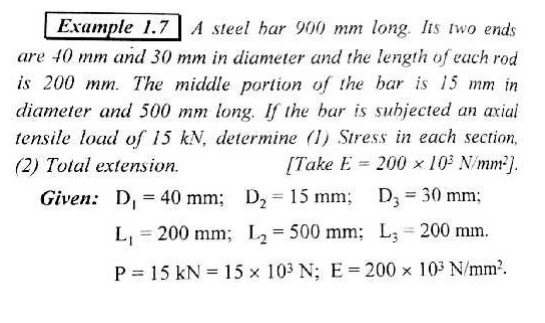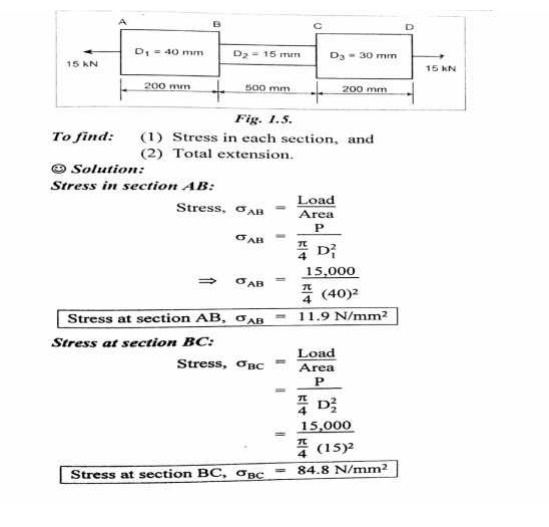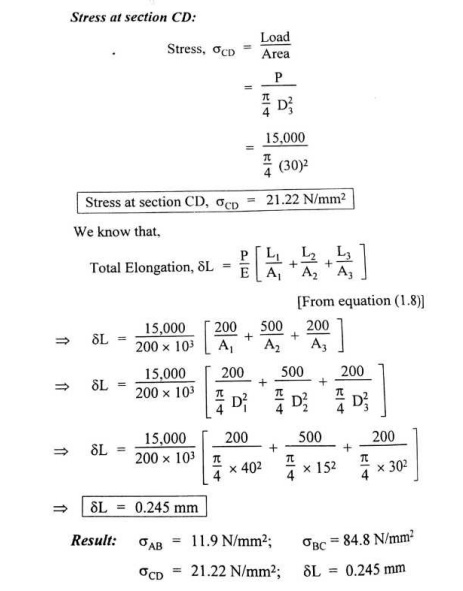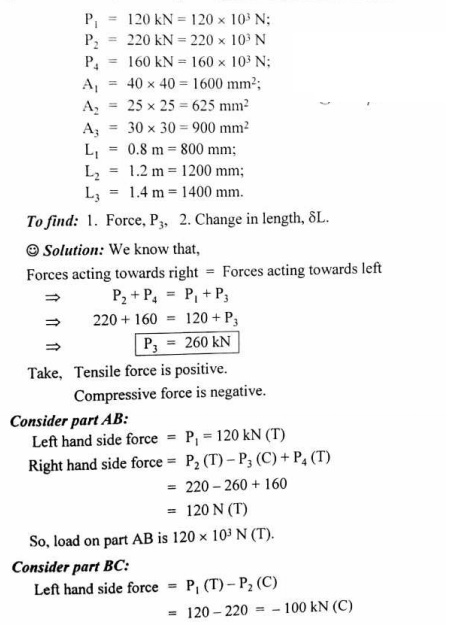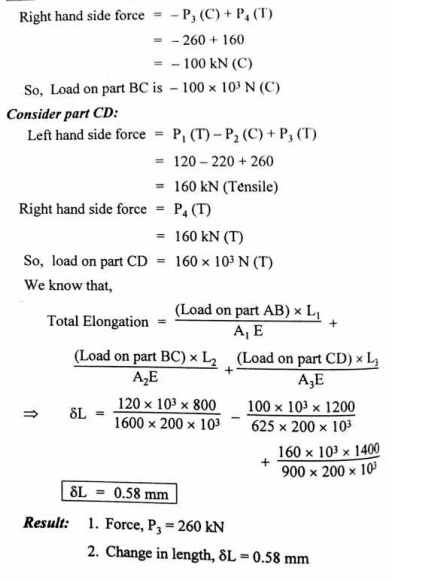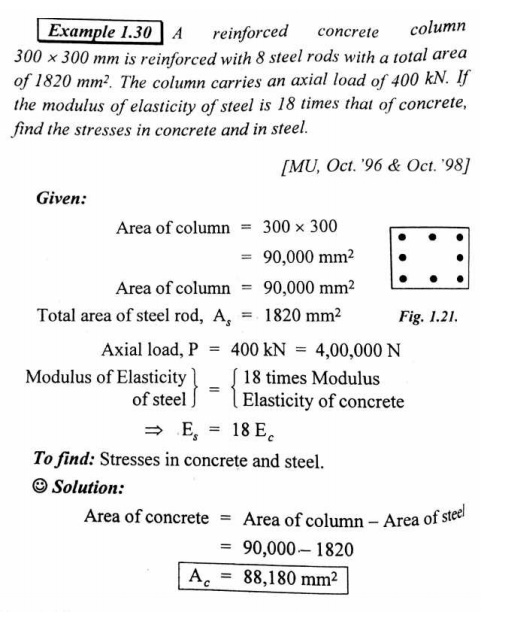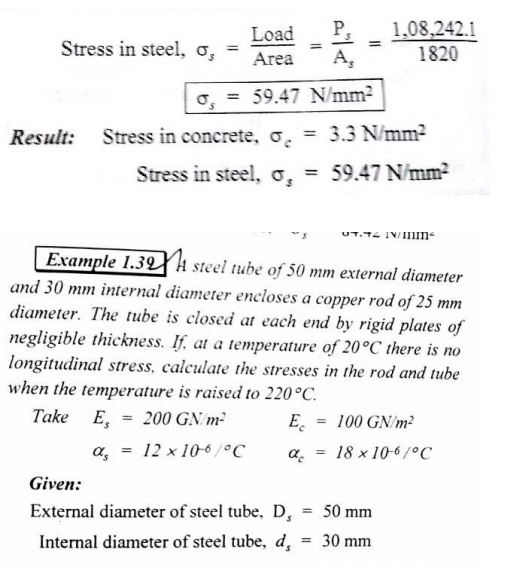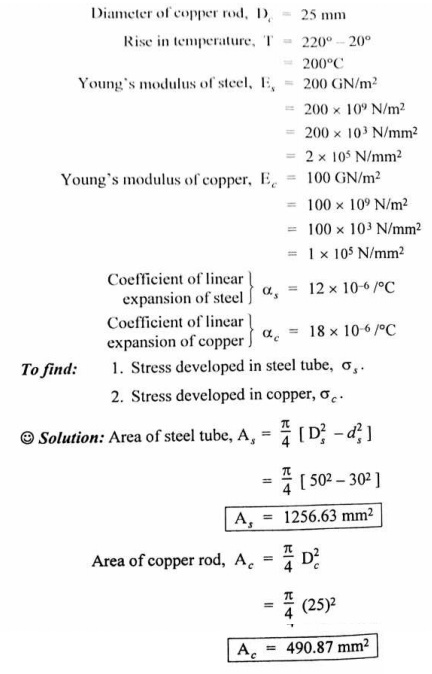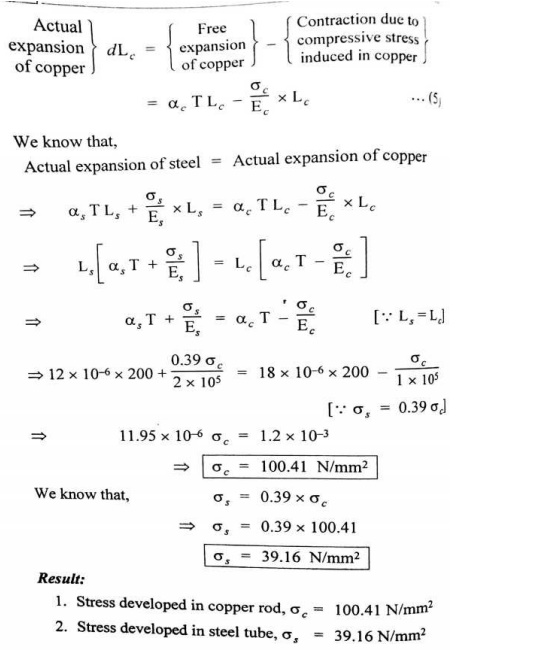Beams are classified on the basis of their geometry and the manner in which they are supported.

Beams classification

Classification of Beams:

Beams are classified on the basis of their geometry and the manner in which they are supported.

Classification I: The classification based on the basis of geometry normally includes features such as the shape of the X-section and whether the beam is straight or curved.

Classification II: Beams are classified into several groups, depending primarily on the kind of supports used. But it must be clearly understood why do we need supports. The supports are required to provide constrainment to the movement of the beams or simply the supports resists the movements either in particular direction or in rotational direction or both. As a consequence of this, the reaction comes into picture whereas to resist rotational movements the moment comes into picture. On the basis of the support, the beams may be classified as follows:

Cantilever Beam: A beam which is supported on the fixed support is termed as a cantilever beam: Now let us understand the meaning of a fixed support. Such a support is obtained by building a beam into a brick wall, casting it into concrete or welding the end of the beam. Such a support provides both the translational and rotational constrainment to the beam, therefore the reaction as well as the moments appears, as shown in the figure below

Simply Supported Beam: The beams are said to be simply supported if their supports creates only the translational constraints.

Some times the translational movement may be allowed in one direction with the help of rollers and can be represented like this

Statically Determinate or Statically Indeterminate Beams:

The beams can also be categorized as statically determinate or else it can be referred as statically indeterminate. If all the external forces and moments acting on it can be determined from the equilibrium conditions alone then. It would be referred as a statically determinate beam, whereas in the statically indeterminate beams one has to consider deformation i.e. deflections to solve the problem.

Types of beams: Supports and Loads

In many engineering structures members are required to resist forces that are applied laterally or transversely to their axes. These type of members are termed as beam. There are various ways to define the beams such as

Definition I: A beam is a laterally loaded member, whose cross-sectional dimensions are small as compared to its length.

Definition II: A beam is nothing simply a bar which is subjected to forces or couples that lie in a plane containing the longitudnal axis of the bar. The forces are understood to act perpendicular to the longitudnal axis of the bar.

Definition III: A bar working under bending is generally termed as a beam.

The beams may be made from several usable engineering materials such commonly among them are as follows: Metal, Wood, Concrete, Plastic.

Materials for Beam:

The beams may be made from several usable engineering materials such commonly among them are as follows:

Metal

Wood

Concrete

Plastic

Issues Regarding Beam:

Designer would be interested to know the answers to following issues while dealing with beams in practical engineering application

�  At what load will it fail

�  How much deflection occurs under the application of loads.

Cantilever Beam: A beam which is supported on the fixed support is termed as a cantilever beam: Now let us understand the meaning of a fixed support. Such a support is obtained by building a beam into a brick wall, casting it into concrete or welding the end of the beam. Such a support provides both the translational and rotational constrainment to the beam, therefore the reaction as well as the moments appears, as shown in the figure below

Simply Supported Beam: The beams are said to be simply supported if their supports creates only the translational constraints.

Some times the translational movement may be allowed in one direction with the help of rollers and can be represented like this

Statically Determinate or Statically Indeterminate Beams:

The beams can also be categorized as statically determinate or else it can be referred as statically indeterminate. If all the external forces and moments acting on it can be determined from the equilibrium conditions alone then. It would be referred as a statically determinate beam, whereas in the statically indeterminate beams one has to consider deformation i.e. deflections to solve the problem.

Types of loads acting on beams:

A beam is normally horizontal where as the external loads acting on the beams is generally in the vertical directions. In order to study the behaviors of beams under flexural loads. It becomes pertinent that one must be familiar with the various types of loads acting on the beams as well as their physical manifestations.

A.  Concentrated Load: It is a kind of load which is considered to act at a point. By this we mean that the length of beam over which the force acts is so small in comparison to its total length that one can model the force as though applied at a point in two dimensional view of beam. Here in this case, force or load may be made to act on a beam by a hanger or though other means

B.  Distributed Load: The distributed load is a kind of load which is made to spread over a entire span of beam or over a particular portion of the beam in some specific manner

In the above figure, the rate of loading �q’ is a function of x i.e. span of the beam, hence this is a non uniformly distributed load.

The rate of loading �q’ over the length of the beam may be uniform over the entire span of beam, then we cell this as a uniformly distributed load (U.D.L). The U.D.L may be represented in either of the way on the beams

some times the load acting on the beams may be the uniformly varying as in the case of dams or on inclind wall of a vessel containing liquid, then this may be represented on the beam as below:

The U.D.L can be easily realized by making idealization of the ware house load, where the bags of grains are placed over a beam.

Concept of Shear Force and Bending moment in beams:

When the beam is loaded in some arbitrarily manner, the internal forces and moments are developed and the terms shear force and bending moments come into pictures which are helpful to analyze the beams further. Let us define these terms

Now let us consider the beam as shown in fig 1(a) which is supporting the loads P1, P2, P3 and is simply supported at two points creating the reactions R1 and R2respectively. Now let us assume that the beam is to divided into or imagined to be cut into two portions at a section AA. Now let us assume that the resultant of loads and reactions to the left of AA is �F’ vertically upwards, and

since the entire beam is to remain in equilibrium, thus the resultant of forces to the right of AA must also be F, acting downwards. This forces �F’ is as a shear force. The shearing force at any x

section of a beam represents the tendency for the portion of the beam to one side of the section to slide or shear laterally relative to the other portion.

Therefore, now we are in a position to define the shear force �F’ to as follows:

At any x-section of a beam, the shear force �F’ is the algebraic sum of all the lateral components of the forces acting on either side of the x-section.

Sign Convention for Shear Force:

The usual sign conventions to be followed for the shear forces have been illustrated in figures 2 and 3.

Bending Moment:

Let us again consider the beam which is simply supported at the two prints, carrying loads P1, P2 and P3 and having the reactions R1 and R2 at the supports Fig 4. Now, let us imagine that the beam is cut into two potions at the x-section AA. In a similar manner, as done for the case of shear force, if we say that the resultant moment about the section AA of all the loads and

reactions to the left of the x-section at AA is M in C.W direction, then moment of forces to the right of x-section AA must be �M’ in C.C.W. Then �M’ is called as the Bending moment and is

abbreviated as B.M. Now one can define the bending moment to be simply as the algebraic sum of the moments about an x-section of all the forces acting on either side of the section

Sign Conventions for the Bending Moment:

For the bending moment, following sign conventions may be adopted as indicated in Fig 5 and Fig 6.

Some times, the terms �Sagging’ and Hogging are generally used for the positive and negative bending moments respectively.

Bending Moment and Shear Force Diagrams:

The diagrams which illustrate the variations in B.M and S.F values along the length of the beam for any fixed loading conditions would be helpful to analyze the beam further.

Thus, a shear force diagram is a graphical plot, which depicts how the internal shear force �F varies along the length of beam. If x dentotes the length of the beam, then F is function x i.e. F(x).

Similarly a bending moment diagram is a graphical plot which depicts how the internal bending moment �M’ varies along the length of the beam. Again M is a function x i.e. M(x).

Basic Relationship Between The Rate of Loading, Shear Force and Bending Moment:

The construction of the shear force diagram and bending moment diagrams is greatly simplified if the relationship among load, shear force and bending moment is established.

Let us consider a simply supported beam AB carrying a uniformly distributed load w/length. Let us imagine to cut a short slice of length dx cut out from this loaded beam at distance �x’ from the origin �0′.

Let us detach this portion of the beam and draw its free body diagram.

The forces acting on the free body diagram of the detached portion of this loaded beam are the following

� The shearing force F and F+ dF at the section x and x + dx respectively.

� The bending moment at the sections x and x + dx be M and M + dM respectively.

�  Force due to external loading, if �w’ is the mean rate of loading per unit length then the total loading on this slice of length dx is w. dx, which is approximately acting through the centre �c’.

If the loading is assumed to be uniformly distributed then it would pass exactly through the centre �c’.

This small element must be in equilibrium under the action of these forces and couples.

Now let us take the moments at the point �c’. Such that

Conclusions: From the above relations,the following important conclusions may be drawn

�  From Equation (1), the area of the shear force diagram between any two points, from the basic calculus is the bending moment diagram

� The slope of bending moment diagram is the shear force,thus

Thus, if F=0; the slope of the bending moment diagram is zero and the bending moment is therefore constant.’

� The maximum or minimum Bending moment occurs where

The slope of the shear force diagram is equal to the magnitude of the intensity of the distributed loading at any position along the beam. The ve sign is as a consequence of our particular choice of sign conventions

Procedure for drawing shear force and bending moment diagram:

Preamble:

The advantage of plotting a variation of shear force F and bending moment M in a beam as a function of �x’ measured from one end of the beam is that it becomes easier to determine the

maximum absolute value of shear force and bending moment.

Further, the determination of value of M as a function of �x‘ becomes of paramount importance so as to determine the value of deflection of beam subjected to a given loading.

Construction of shear force and bending moment diagrams:

A shear force diagram can be constructed from the loading diagram of the beam. In order to draw this, first the reactions must be determined always. Then the vertical components of forces and reactions are successively summed from the left end of the beam to preserve the mathematical sign conventions adopted. The shear at a section is simply equal to the sum of all the vertical forces to the left of the section.

When the successive summation process is used, the shear force diagram should end up with the previously calculated shear (reaction at right end of the beam. No shear force acts through the beam just beyond the last vertical force or reaction. If the shear force diagram closes in this fashion, then it gives an important check on mathematical calculations.

The bending moment diagram is obtained by proceeding continuously along the length of beam from the left hand end and summing up the areas of shear force diagrams giving due regard to sign. The process of obtaining the moment diagram from the shear force diagram by summation is exactly the same as that for drawing shear force diagram from load diagram.

It may also be observed that a constant shear force produces a uniform change in the bending moment, resulting in straight line in the moment diagram. If no shear force exists along a certain portion of a beam, then it indicates that there is no change in moment takes place. It may also further observe that dm/dx= F therefore, from the fundamental theorem of calculus the maximum or minimum moment occurs where the shear is zero. In order to check the validity of the bending moment diagram, the terminal conditions for the moment must be satisfied. If the end is free or pinned, the computed sum must be equal to zero. If the end is built in, the moment computed by the summation must be equal to the one calculated initially for the reaction. These conditions must always be satisfied.

Cantilever beams – problems

Cantilever with a point load at the free end:Mx = – w.x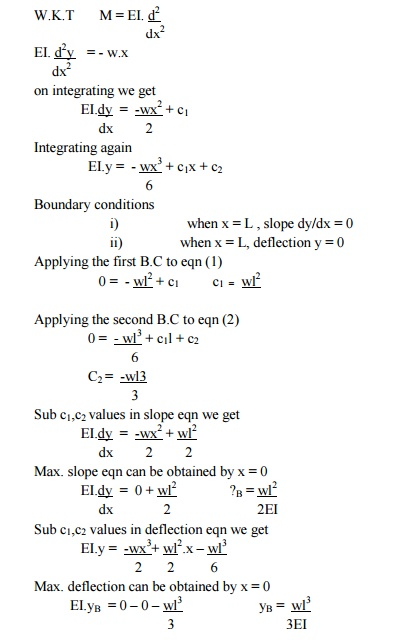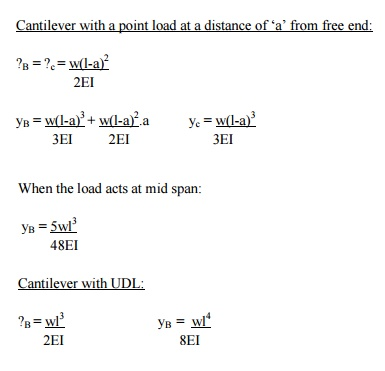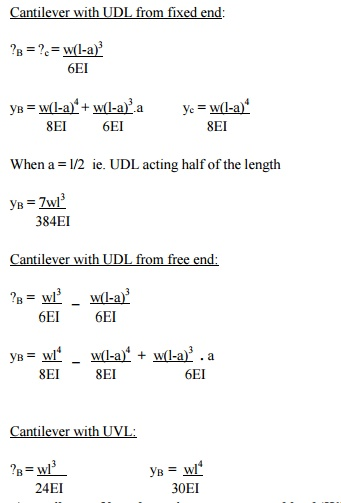A cantilever of length carries a concentrated load ‘W’ at its free end.

Draw shear force and bending moment.

Solution:

At a section a distance x from free end consider the forces to the left, then F = -W (for all values of x) -ve sign means the shear force to the left of the x-section are in downward direction and therefore negative

Taking moments about the section gives (obviously to the left of the section)

M = -Wx (-ve sign means that the moment on the left hand side of the portion is in the anticlockwise direction and is therefore taken as ve according to the sign convention)

so that the maximum bending moment occurs at the fixed end i.e. M = -W l

Simplysupported beam -problems

Simply supported beam subjected to a central load (i.e. load acting at the mid-way)

By symmetry the reactions at the two supports would be W/2 and W/2. now consider any section X-X from the left end then, the beam is under the action of following forces.

.So the shear force at any X-section would be = W/2 [Which is constant upto x < l/2]

If we consider another section Y-Y which is beyond l/2 then

for all values greater = l/2

SSB with central point load: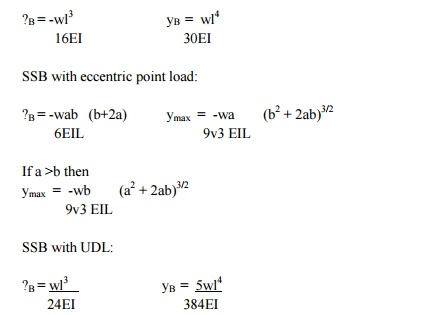Overhanging beams – problems

In the problem given below, the intensity of loading varies from q1 kN/m at one end to the q2 kN/m at the other end.This problem can be treated by considering a U.d.i of intensity q1 kN/m over the entire span and a uniformly varying load of 0 to ( q2- q1)kN/m over the entire span and then super impose teh two loadings.

Point of Contraflexure:

Consider the loaded beam a shown below along with the shear force and Bending moment diagrams for It may be observed that this case, the bending moment diagram is completely positive so that the curvature of the beam varies along its length, but it is always concave upwards or sagging.However if we consider a again a loaded beam as shown below along with the S.F and B.M diagrams, then

It may be noticed that for the beam loaded as in this case,

The bending moment diagram is partly positive and partly negative.If we plot the deflected shape of the beam just below the bending moment

This diagram shows that L.H.S of the beam �sags’ while the R.H.S of the beam �hogs’

The point C on the beam where the curvature changes from sagging to hogging is a point of contraflexure.

OR

It corresponds to a point where the bending moment changes the sign, hence in order to find the point of contraflexures obviously the B.M would change its sign when it cuts the X-axis therefore to get the points of contraflexure equate the bending moment equation equal to zero.The fibre stress is zero at such sections

Note: there can be more than one point of contraflexure

Preamble:

When a beam having an arbitrary cross section is subjected to a transverse loads the beam will bend. In addition to bending the other effects such as twisting and buckling may occur, and to investigate a problem that includes all the combined effects of bending, twisting and buckling could become a complicated one. Thus we are interested to investigate the bending effects alone, in order to do so, we have to put certain constraints on the geometry of the beam and the manner of loading.

Assumptions:

The constraints put on the geometry would form the assumptions:

1. Beam is initially straight , and has a constant cross-section.

2.    Beam is made of homogeneous material and the beam has a longitudinal plane of symmetry.

3. Resultant of the applied loads lies in the plane of symmetry.

4. The geometry of the overall member is such that bending not buckling is the primary cause of failure.

5. Elastic limit is nowhere exceeded and ‘E’ is same in tension and compression.

6. Plane cross – sections remains plane before and after bending.

Let us consider a beam initially unstressed as shown in fig 1(a). Now the beam is subjected to a constant bending moment (i.e. �Zero Shearing Force’) along its length as would be obtained by

applying equal couples at each end. The beam will bend to the radius R as shown in Fig 1(b)

As a result of this bending, the top fibers of the beam will be subjected to tension and the bottom to compression it is reasonable to suppose, therefore, that some where between the two there are points at which the stress is zero. The locus of all such points is known as neutral axis . The radius of curvature R is then measured to this axis. For symmetrical sections the N. A. is the axis of symmetry but what ever the section N. A. will always pass through the centre of the area or centroid.

As we are aware of the fact internal reactions developed on any cross-section of a beam may consists of a resultant normal force, a resultant shear force and a resultant couple. In order to ensure that the bending effects alone are investigated, we shall put a constraint on the loading such that the resultant normal and the resultant shear forces are zero on any cross-section perpendicular to the longitudinal axis of the member, That means F = 0 since or M = constant.

Thus, the zero shear force means that the bending moment is constant or the bending is same at every cross-section of the beam. Such a situation may be visualized or envisaged when the beam

or some portion of the beam, as been loaded only by pure couples at its ends. It must be recalled that the couples are assumed to be loaded in the plane of symmetry.

When a member is loaded in such a fashion it is said to be in pure bending. The examples of pure bending have been indicated in EX 1and EX 2 as shown below :

When a beam is subjected to pure bending are loaded by the couples at the ends, certain cross- section gets deformed and we shall have to make out the conclusion that,

1.  Plane sections originally perpendicular to longitudinal axis of the beam remain plane and perpendicular to the longitudinal axis even after bending , i.e. the cross-section A’E’, B’F’ ( refer Fig 1(a) ) do not get warped or curved.

2. In the deformed section, the planes of this cross-section have a common intersection i.e. any time originally parallel to the longitudinal axis of the beam becomes an arc of circle.

We know that when a beam is under bending the fibres at the top will be lengthened while at the bottom will be shortened provided the bending moment M acts at the ends. In between these there are some fibres which remain unchanged in length that is they are not strained, that is they do not carry any stress. The plane containing such fibres is called neutral surface.

The line of intersection between the neutral surface and the transverse exploratory section is called the neutral axisNeutral axis (N A) .

Bending Stresses in Beams or Derivation of Elastic Flexural formula :

In order to compute the value of bending stresses developed in a loaded beam, let us consider the two cross-sections of a beam HE and GF , originally parallel as shown in fig 1(a).when the beam

is to bend it is assumed that these sections remain parallel i.e. H’E’ and G’F’ , the final position of the sections, are still straight lines, they then subtend some angle q.

Consider now fiber AB in the material, at adistance y from the N.A, when the beam bends this will stretch to A’B’

Since CD and C’D’ are on the neutral axis and it is assumed that the Stress on the neutral axis zero. Therefore, there won’t be any strain on the neutral axis

Consider any arbitrary a cross-section of beam, as shown above now the strain on a fibre at a distance �y’ from the N.A, is given by the expression

Now the termis the property of the material and is called as a second moment of area of the cross-section and is denoted by a symbol I.

Therefore  M/I = sigma/y = E/R

This equation is known as the Bending Theory Equation.The above proof has involved the assumption of pure bending without any shear force being present. Therefore this termed as the pure bending equation. This equation gives distribution of stresses which are normal to cross-section i.e. in x-direction.

Stress variation along the length and in the beam section

Bending Stress and Deflection Equation

In this section, we consider the case of pure bending; i.e., where only bending stresses exist as a

result of applied bending moments. To develop the theory, we will take the phenomenological approach to develop what is called the ‘Euler-Bernoulli theory of beam bending.’ Geometry:

Consider a long slender straight beam of length L and cross-sectional area A. We assume the beam is prismatic or nearly so. The length dimension is large compared to the dimensions of the cross-section. While the cross-section may be any shape, we will assume that it is symmetric about the y axis

Loading: For our purposes, we will consider shear forces or distributed loads that are applied in the y direction only (on the surface of the beam) and moments about the z-axis. We have consider examples of such loading in ENGR 211 previously and some examples are shown below:

Kinematic Observations: In order to obtain a ‘feel’ for the kinematics (deformation) of a beam

subjected to pure bending loads, it is informative to conduct an experiment. Consider a rectangular lines have been scribed on the beam’s surface, which are parallel to the top and

bottom surfaces (and thus parallel to a centroidally placed x-axis along the length of the beam). Lines are also scribed around the circumference of the beam so that they are perpendicular to the longitudinals (these circumferential lines form flat planes as shown). The longitudinal and circumferential lines form a square grid on the surface. The beam is now bent by moments at each end as shown in the lower photograph. After loading, we note that the top line has stretched and the bottom line has shortened (implies that there is strain exx). If measured carefully, we see that the longitudinal line at the center has not changed length (implies that exx = 0 at y = 0). The longitudinal lines now appear to form concentric circular lines.

We also note that the vertical lines originally perpendicular to the longitudinal lines remain straight

and perpendicular to the longitudinal lines. If measured carefully, we will see that the vertical lines remain approximately the same length (implies eyy = 0). Each of the vertical lines (as well as the planes they form) has rotated and, if extended downward, they will pass through a common point that forms the center of the concentric longitudinal lines (with some radius ?). The flat planes originally normal to the longitudinal axis remain essentially flat planes and remain normal to the deformed longitudinal lines. The squares on the surface are now quadrilaterals and each appears to have tension (or compression) stress in the longitudinal direction (since the horizontal lines of a square have changed length). However, in pure bending we make the assumption that. If the x-axis is along the length of beam and the y-axis is normal to the beam, this suggests that we have an axial normal stress sxx that is tension above the x-axis and compression below the y-axis. The remaining normal stresses syy and szz will generally be negligible for pure bending about the z-axis. For pure bending, all shear stresses are assumed to be zero. Consequently, for pure bending, the stress matrix reduces to zero.

CIRCULAR SECTION :

For a circular x-section, the polar moment of inertia may be computed in the following manner

Consider any circular strip of thickness dr located at a radius ‘r’.

Than the area of the circular strip would be dA = 2pr. dr

Thus

Parallel Axis Theorem:

The moment of inertia about any axis is equal to the moment of inertia about a parallel axis through the centroid plus the area times the square of the distance between the axes.

If �ZZ’ is any axis in the plane of crosssection and �XX’ is a parallel axis through the centroid G, of the cross-section, then

Rectangular Section:

For a rectangular x-section of the beam, the second moment of area may be computed as below :

Consider the rectangular beam cross-section as shown above and an element of area dA , thickness dy , breadth B located at a distance y from the neutral axis, which by symmetry passes through the centre of section. The second moment of area I as defined earlier would be

Thus, for the rectangular section the second moment of area about the neutral axis i.e., an axis through the centre is given by

Similarly, the second moment of area of the rectangular section about an axis through the lower edge of the section would be found using the same procedure but with integral limits of 0 to D .

Therefore

These standards formulas prove very convenient in the determination of INA for build up sections which can be conveniently divided into rectangles. For instance if we just want to find out the Moment of Inertia of an I – section, then we can use the above relation.

Let us consider few examples to determaine the sheer stress distribution in a given X-sections

Rectangular x-section:

Consider a rectangular x-section of dimension b and d

A is the area of the x-section cut off by a line parallel to the neutral axis. is the distance of the centroid of A from the neutral axis

This shows that there is a parabolic distribution of shear stress with y.

The maximum value of shear stress would obviously beat the location y = 0.

Therefore the shear stress distribution is shown as below.

It may be noted that the shear stress is distributed parabolically over a rectangular cross-section, it is maximum at y = 0 and is zero at the extreme ends.

I – section :

Consider an I – section of the dimension shown below.

The shear stress distribution for any arbitrary shape is given as

Let us evaluate the quantity, thequantity for this case comprise the contribution due to flange area and web area

Flange area

Web Area

To get the maximum and minimum values of t substitute in the above relation.

y = 0 at N. A. And y = d/2 at the tip.

The maximum shear stress is at the neutral axis. i.e. for the condition y = 0 at N. A.

Hence, ……….(2)

The minimum stress occur at the top of the web, the term bd 2 goes off and shear stress is given by the following expression

…………(3)

The distribution of shear stress may be drawn as below, which clearly indicates a parabolic distribution

Note: from the above distribution we can see that the shear stress at the flanges is not zero, but it has some value, this can be analyzed from equation (1). At the flange tip or flange or web interface y = d/2.Obviously than this will have some constant value and than onwards this will have parabolic distribution.

In practice it is usually found that most of shearing stress usually about 95% is carried by the web, and hence the shear stress in the flange is neglible however if we have the concrete analysis i.e. if we analyze the shearing stress in the flange i.e. writing down the expression for shear stress for flange and web separately, we will have this type of variation.

This distribution is known as the “top  hat” distribution. Clearly the web bears the most of the shear stress and bending theory we can say that the flange will bear most of the bending stress.

Shear stress distribution in beams of circular cross-section:

Let us find the shear stress distribution in beams of circular cross-section. In a beam of circular cross-section, the value of Z width depends on y.

Using the expression for the determination of shear stresses for any arbitrary shape or a arbitrary section.

Where �y dA is the area moment of the shaded portion or the first moment of area.

Here in this case �dA’ is to be found out using the Pythagoras theorem

The distribution of shear stresses is shown below, which indicates a parabolic distribution

Principal Stresses in Beams

It becomes clear that the bending stress in beam sx is not a principal stress, since at any distance y from the neutral axis; there is a shear stress t ( or txy we are assuming a plane stress situation)

In general the state of stress at a distance y from the neutral axis will be as follows.

At some point �P’ in the beam, the value of bending stresses is given as

After substituting the appropriate values in the above expression we may get the inclination of the principal planes.

Illustrative examples: Let us study some illustrative examples,pertaining to determination of principal stresses in a beam

1.     Find the principal stress at a point A in a uniform rectangular beam 200 mm deep and 100 mm wide, simply supported at each end over a span of 3 m and carrying a uniformly distributed load of 15,000 N/m.

Solution: The reaction can be determined by symmetry

R1 = R2 = 22,500 N

consider any cross-section X-X located at a distance x from the left end.

Hence,

S. F at XX =22,500  15,000 x

B.M at XX = 22,500 x  15,000 x (x/2) = 22,500 x  15,000 . x2 / 2

Therefore,

S. F at X = 1 m = 7,500 N

B. M at X = 1 m = 15,000 N

Now substituting these values in the principal stress equation,

We get s1 = 11.27 MN/m2

s2 = – 0.025 MN/m2

Bending Of Composite or Flitched Beams

A composite beam is defined as the one which is constructed from a combination of materials. If such a beam is formed by rigidly bolting together two timber joists and a reinforcing steel plate, then it is termed as a flitched beam.

The bending theory is valid when a constant value of Young’s modulus applies across a section it cannot be used directly to solve the composite-beam problems where two different materials, and therefore different values of E, exists. The method of solution in such a case is to replace one of the materials by an equivalent section of the other.

Consider, a beam as shown in figure in which a steel plate is held centrally in an appropriate recess/pocket between two blocks of wood .Here it is convenient to replace the steel by an equivalent area of wood, retaining the same bending strength. i.e. the moment at any section must be the same in the equivalent section as in the original section so that the force at any given dy in the equivalent beam must be equal to that at the strip it replaces.

Hence to replace a steel strip by an equivalent wooden strip the thickness must be multiplied by the modular ratio E/E’.

The equivalent section is then one of the same materials throughout and the simple bending

theory applies. The stress in the wooden part of the original beam is found directly and that in the steel found from the value at the same point in the equivalent material as follows by utilizing the given relations.

Stress in steel = modular ratio x stress in equivalent wood

The above procedure of course is not limited to the two materials treated above but applies well for any material combination. The wood and steel flitched beam was nearly chosen as a just for the sake of convenience.

Assumption

In order to analyze the behavior of composite beams, we first make the assumption that the materials are bonded rigidly together so that there can be no relative axial movement between them. This means that all the assumptions, which were valid for homogenous beams are valid except the one assumption that is no longer valid is that the Young’s Modulus is the same throughout the beam.

The composite beams need not be made up of horizontal layers of materials as in the earlier example. For instance, a beam might have stiffening plates as shown in the figure below.

Again, the equivalent beam of the main beam material can be formed by scaling the breadth of the plate material in proportion to modular ratio. Bearing in mind that the strain at any level is same in both materials, the bending stresses in them are in proportion to the Young’s modulus.

Shear stresses in beams

When a beam is subjected to nonuniform bending, both bending moments, M, and shear forces, V, act on the cross section. The normal stresses, sx, associated with the bending moments are obtained from the flexure formula. We will now consider the distribution of shear stresses, t, associated with the shear force, V. Let us begin by examining a beam of rectangular cross section. We can reasonably assume that the shear stresses t act parallel to the shear force V. Let us also assume that the distribution of shear stresses is uniform across the width of the beam.

Shear flow

One thing we might ask ourselves now is: Where does maximum shear stress occur? Well, it can be shown that this always occurs in the center of gravity of the cross-section. So if you want to calculate the maximum shear stress, make a cut through the center of gravity of the cross-section.

Civil – Mechanics Of Solids – Transverse Loading On Beams And Stresses In Beam

TRANSVERSE LOADING ON BEAMS AND STRESSES IN BEAMS

1. What is beam?

Beam is structural member which is supported along the length and subjected to external forces acting transversely to the centre line.

2.  Define Shear force

Shear force at a section in a beam is defined as the algebraic sum of all the vertical forces acting on the beam either to the left (or) to the right of the section.

3.  Define Bending moment.

Bending moment at a section in a beam is defined as the algebraic sum of moments about the section of the beam either to the left (or) to the right of the section.

4.  Write four types of beams based on their types of supports?

a. Simply supported beams                b. Fixed beam

c. Cantilever beam                             d. Propped cantilever beam

5.  What are the different types of loading on a beam?

a. Concentrate load (or) point load   b. Uniformly distributed load

c. Non-uniform distributed load        d. Couple or moment.

6.     What are the different types of supports?

(i) Simply support (or) roller support

(ii) Pinned support (or) Hinged support

(iii) Rigid support (or) Fixed support

7.     What is a simply supported beam?

A beam supported or resting freely on the walls columns at its both ends is known as a simply supported beam.

8.     What is Cantilever beam?

A beam fixed at one end and free at the other end is known as a cantilever beam.

9.  What is continuous beam?

A beam which has more than two supports is called continuous beam.

10. What is overhanging beam?

If the one or both the end portions are extended beyond the support then it is called.

11. Define fixed beams.

A beam whose both ends are rigidly fixed or built-in walls is known as rigidly fixed beam or a built in beam.

12. What is transverse load?

A load which has more than two supports is called transverse load.

13. What is point load?

A load which is acting at a particular point is called point load.

14. What is uniformly distributed load?

A load which is spread over a beam in such a manner that the rate of loading ‘w’ is uniform throughout the length.

15. What is shear force?

It is the unbalanced vertical forces on the left or right section of the beam.

16. Define bending moment.

Bending at a cross section is the algebraic sum of the moments of all the forces which are placed either side from that point.

17. Write the relation between SF and BM.

The rate of change of BM is equal to the SF at the section dM/dx = – F

18.  A  clockwise  moment  M  is  applied  at     the  free  end  of  cantilever.  Draw  the  SF  and  BM diagrams of the cantilever.

SF Calculation     SF diagram

SFA= 0

SF at B = 0

BM Calculation    BM diagram

BM at B = -M

(Since for counterclockwise moment)

BM at A = -M

(BM at both the ends is same)

19. A simply supported beam of span ‘l’ is subjected to central concentrated load ‘W’. What is the bending moment at the centre?

Bending moment at the centre Mc = Wl/4

20.            What is point of contra flexure? Whether point of contra flexure will occur in a cantilever beam?

The point where BM is zero after changing its sign is known as point of contra flexure.

21.            Whether point of contra flexure will occur in a cantilever beam?

The Point of contra flexure will not occur in a cantilever beam.

22. Define pure bending (or) bending stress.

Simple bending means flexure by pure bending moment without shear force.

23.            What are the assumptions are made in the theory of pure bending?

a)    The value of the young’s modulus is the same for the beam material in tension as well as compression.

b)    The transverse section of the beam, which is a plane before bending, will remain a plane after bending.

c)     The material of the beam is homogeneous and isotropic.

24.            State the theory of simple bending.

If a beam is bent only due to application of constant BM and not due to shear then it is called simple bending.

25. Write down the bending equation.

= f = E I y RM – Moment of resistance I – Moment of inertia

f – Maximum bending stress

y – Distance from the neutral axis E – Young’s modulus

R – Radius of the curvature

26. Define Section modulus

It is the ratio of moment of inertia about the neutral axis to distance of the extreme fiber from the neutral axis.

Section modulus Z = I / Y

27.  What is moment of resistance of the section?

It is the product of section modulus and bending stress at that section.

M = f x Z

28. What is a flitched beam? Why it is used?

A flitched beam means a beam of composite section consisting of a wooden beam strength ended by mild steel plates. It is mainly used to reinforce the material which has lower strength and reduce the cost.

29. Why flitched beam is used?

It is mainly used to reinforce the material which has lower strength and reduce the cost.

30. What is the value of maximum shear stress in a rectangular cross section?

The max shear stress in a rectangular section is 1.5 times the average stress.

31.  Write  down  the  equation  for  shear  stress  distribution  across  a  circular  section  with  radius.

R at a distance y from neutral axis.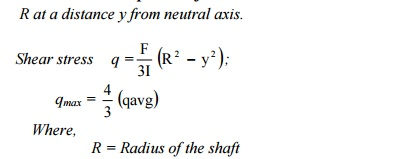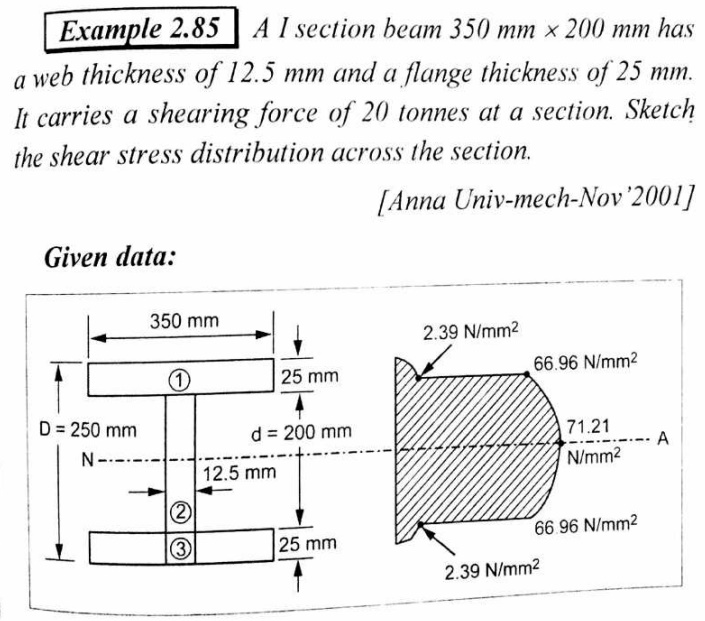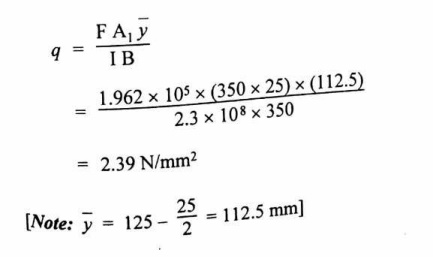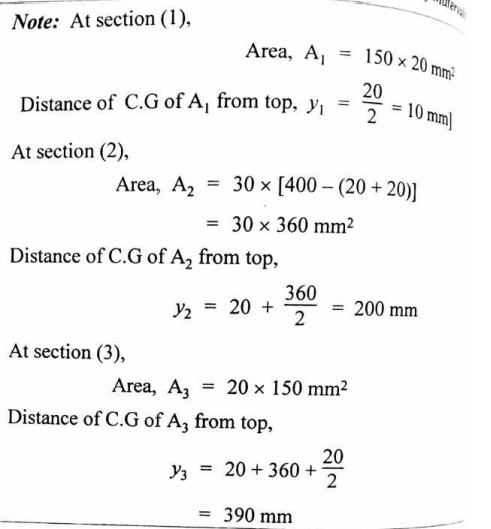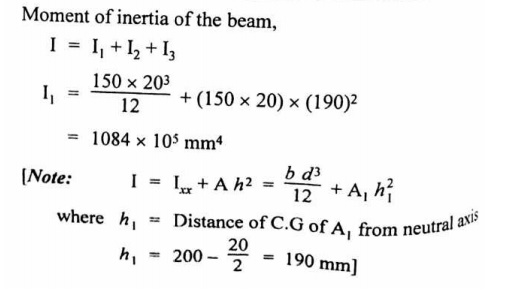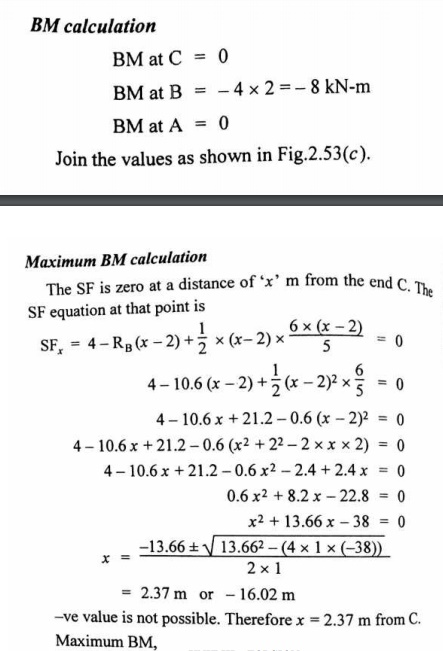In solid mechanics, torsion is the twisting of an object due to an applied torque. In sections perpendicular to the torque ax is, the resultant shear stress in this section is perpendicular to the radius.

For solid shafts of uniform circular cross-section or hollow circular shaf ts with constant wall thickness, the torsion relations are: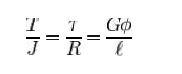where:

�        R is the outer radius of the shaft i.e. m, ft.

�        t is the maximum shear stress at the outer surface.

�        is the angle of twist in radians.

�        is the torque (N�m or ft�lbf).

�        is the length of the object the torque is being applied to or over.

�        is the shear modulus or more commonly the modulus of rigidity and is usually given

in gigapascals (GPa), lb f/in2 (psi), or lbf/ft2.

�        is the torsion constant for the section. It is identical to the polar moment of inertia for a round shaft or concentric tube only. For other shapes J must be determined by other means. For solid shafts the membrane analogy is useful, and for thin walled tubes of arbitrary shape the she ar flow approximation is fairly good, if t he section is not re-entrant. For thick walle d tubes of arbitrary shape there is no simp le solution, and finite element analysis (FEA) may be the best method.

�        The product GJ is calle d the torsional rigidity.